Theory and Modern Applications

# An observer-based vaccination control law for an SEIR epidemic model based on feedback linearization techniques for nonlinear systems

## Abstract

This paper presents a vaccination strategy for fighting against the propagation of epidemic diseases. The disease propagation is described by an SEIR (susceptible plus infected plus infectious plus removed populations) epidemic model. The model takes into account the total population amounts as a refrain for the illness transmission since its increase makes the contacts among susceptible and infected more difficult. The vaccination strategy is based on a continuous-time nonlinear control law synthesised via an exact feedback input-output linearization approach. An observer is incorporated into the control scheme to provide online estimates for the susceptible and infected populations in the case when their values are not available from online measurement but they are necessary to implement the control law. The vaccination control is generated based on the information provided by the observer. The control objective is to asymptotically eradicate the infection from the population so that the removed-by-immunity population asymptotically tracks the whole one without precise knowledge of the partial populations. The model positivity, the eradication of the infection under feedback vaccination laws and the stability properties as well as the asymptotic convergence of the estimation errors to zero as time tends to infinity are investigated.

## 1 Introduction

A relevant area in the mathematical theory of epidemiology is the development of models for studying the propagation of epidemic diseases in a host population . The epidemic mathematical models analysed in such an exhaustive list of books and papers include the most basic ones , namely (i) SI models where only susceptible and infected populations are assumed to be present in the model, (ii) SIR models which include susceptible plus infected plus removed-by-immunity populations and (iii) SEIR models where the infected population is split into two ones, namely the ‘infected’ (or ‘exposed’) which incubate the disease but do not still have any disease symptoms and the ‘infectious’ (or ‘infective’) which do have the external disease symptoms. Those models can be divided into two main classes, namely the so-called ‘pseudo-mass action models’, where the total population is not taken into account as a relevant disease contagious factor and the so-called ‘true-mass action models’, where the total population is more realistically considered as an inverse factor of the disease transmission rates. There are many variants of the above models as, for instance, the SVEIR epidemic models which incorporate the dynamics of a vaccinated population in comparison with the SEIR models  and the SEIQR-SIS model which adds a quarantine population . Other variant consists of the generalisation of such models by incorporating point and/or distributed delays [8, 1012, 14]. All of the aforementioned models are so-called compartmental models since host individuals are classified depending on their status in relation to the infectious disease. However, there are diseases where some factors such as the disease transmission, the mortality rate and so on are the functions of age. Then such diseases are described more precisely by means of the so-called compartmental models with age structure . Moreover, although the dynamics of infectious diseases transmission through a host population is continuous-time, some researchers have proposed models composed of difference equations to describe the dynamics of epidemics and develop treatments to minimise its effects within the population . On the one hand, a key point in such research works is the choice of an optimal-time step in order to obtain an acceptable discrete-time model from the discretisation of the continuous-time ones. On the other hand, an advantage is that discrete-time models are easier to analyse than continuous-time ones, and then the effectiveness of a potential treatment to eradicate the disease from the host population can be easier to derive.

The analysis of the existence of equilibrium points, relative to either the persistence (endemic equilibrium point) or extinction (disease-free equilibrium point) of the epidemics in the host population [6, 9, 1114], the constraints for guaranteeing the positivity and the boundedness of the solutions of such models [11, 12, 17] and the conditions that generate an oscillatory behaviour in such solutions [11, 18] have been some of the main objectives in the literature about epidemic mathematical models. Other important aim is that relative to the design of control strategies in order to eradicate the persistence of the infection in the host population [2, 5, 11, 12, 17]. In this context, an explicit vaccination function of many different kinds may be added to all aforementioned epidemic models, namely constant [5, 12], continuous-time [2, 17], impulsive , mixed constant/impulsive , mixed continuous-time/impulsive , discrete-time and so on. Concretely, the research in  exhaustively analyses the equilibrium points of an SEIR epidemic model under a vaccination strategy based on a state feedback control law with respect to the model parameters and/or the controller gains. The conditions for the eradication of the diseases from the host population, the extinction of the host population or the persistence of the disease in a non-extinguished host population are derived form such a study. Other alternative approaches, as those based on fuzzy rules  or networks framework [13, 20], have been also proposed for modelling the epidemics transmission through a host population. In this way, the influence of certain social network parameters such as visiting probability, hub radius and contact radius on the epidemics propagation has been investigated . Moreover, there are studies about the influence of the immigration on the persistence or extinction of the epidemics in a population subject to immigration from other regions . Also, the influence of epidemic diseases on the dynamics of prey-predator models has been considered in ecoepidemic models .

In this paper, an SEIR epidemic model which includes susceptible (S), infected or exposed (E), infectious (I) and removed-by-immunity (R) populations is considered. The dynamics of susceptible and immune populations are directly affected by a vaccination function $V\left(t\right)$, which also has an indirect influence on the time evolution of exposed and infectious population. In fact, such a vaccination function has to be suitably designed in order to eradicate the infection from the population. This model has already been studied in  from the viewpoint of equilibrium points in the controlled and free-vaccination cases. A vaccination auxiliary control law proportional to the susceptible population was proposed in order to achieve the whole population being asymptotically immune. Such an approach assumed that the SEIR model was of the aforementioned true-mass action type, its parameters were known and the illness transmission was not critical. Moreover, some important issues of positivity, stability and tracking of the SEIR model were discussed. The main drawback of such a control strategy is the need of online measures of the susceptible, infected, infectious and immune populations. However, the precise online measures of susceptible and infected populations are not always feasible in some real situations, while only true measures of the infectious and whole populations are available. The main motivation of the present paper is to provide a control solution to overcome such a drawback. In this sense, the use of a switching control law coupled with a state observer to synthesise the vaccination function under no precise knowledge of the exact partial populations which are online estimated by the observer is proposed. Such a law only switches once and in this way the control process is divided into two stages. In the first stage, the so-called observation stage, the control function is identically zero and only the observer is working to reduce the initial difference between the true infectious population measure and the estimated one provided by the own observer below a prescribe threshold. In the second stage, related to a combined observation/control stage, the vaccination function is synthesised by means of an input-output exact feedback linearization technique while the observer is maintained active providing the estimates of the true partial populations. In both stages, the state observer provides online estimations of susceptible and infected populations through time overcoming the unfeasibility of obtaining true measures of such partial populations. Such a combination of a linearization control strategy with a nonlinear observer to online estimate all the partial populations constitutes the main contribution of the paper. Moreover, mathematical proofs about the epidemics eradication based on such a controlled SEIR model coupled with the nonlinear observer are presented while maintaining the non-negativity of all the partial populations for all time. The exact feedback linearization can be implemented by using a proper nonlinear coordinate transformation and a static-state feedback control. The use of such a linearization strategy is motivated by three main facts, namely (i) it is a power tool for controlling nonlinear systems which is based on well-established technical principles [22, 23], (ii) the given SEIR model is highly nonlinear and (iii) such a control strategy has not been yet applied in epidemic models.

On the one hand, approaches based on switching control laws have been broadly dealt with in the control theory and its applications . On the other hand, the combination of exact feedback linearization techniques with state observers has been widely used in many control applications, for instance, in biological systems and chemical engineering [25, 26]. The exact linearization technique requires the system to satisfy some structural and regularity conditions, like the existence of relative degree, the minimum phase property and the integrability condition [27, 28]. The SEIR epidemic model satisfies such assumptions, and the aforementioned linearization technique can be applied without any modification. Otherwise, alternative approaches developed to approximately linearize nonlinear systems violating one or more of such assumptions could be used [29, 30].

The paper is organised as follows. Section 2 describes the set of differential equations which compound the SEIR model for the propagation of an epidemic disease through a host population. A result related to the positivity property of such a model is proven. Section 3 presents a control action based on an input-output linearization technique, guaranteeing the positivity and stability properties of the system while asymptotically achieving the eradication of the infection from the host population and, simultaneously, the whole population becoming immune. The positivity property is required from the own nature of the system which forbids the existence of negative populations at any time instant. The control strategy requires the knowledge of the susceptible, infected, infectious and whole population for all time. In this context, the knowledge of the infectious and whole population for all time is feasible, but the knowledge of the susceptible and infected population for all time is not a realistic assumption. As a consequence, such partial populations have to be estimated by means of an observer dynamic system. Then a control action based on such estimates, instead of the corresponding true partial populations, is carried out in Section 4. These theoretical results and the effectiveness of the feedback input-output linearizing controller combined with the observer are illustrated by means of some simulation results in Section 5.

Notation ${\mathbb{R}}_{+}^{n}$ is the first open n th real orthant and ${\mathbb{R}}_{0+}^{n}$ is the first closed n th real orthant. $x\in {\mathbb{R}}_{0+}^{n}$ is a positive real n- vector in the usual sense that all its components are non-negative. Also, ${\mathbb{R}}_{+}$ and ${\mathbb{R}}_{0+}$ are, respectively, used instead of ${\mathbb{R}}_{0}^{1}$ and ${\mathbb{R}}_{0+}^{1}$ for scalars. ${I}_{n}\in {\mathbb{R}}^{n×n}$ denotes the identity matrix and $Det\left(M\right)$ the determinant of the matrix M.

## 2 SEIR epidemic model

Let $S\left(t\right)$, $E\left(t\right)$, $I\left(t\right)$ and $R\left(t\right)$ be, respectively, the susceptible, infected (or exposed), infectious and removed-by-immunity populations at time t. Consider a time-invariant true-mass action type SEIR epidemic model given by the following equations: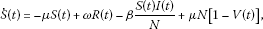(2.1)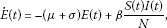(2.2)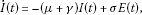(2.3)(2.4)

subject to initial conditions $S\left(0\right)\ge 0$, $E\left(0\right)\ge 0$, $I\left(0\right)\ge 0$ and $R\left(0\right)\ge 0$ under a vaccination function $V:{\mathbb{R}}_{0+}\to {\mathbb{R}}_{0+}$. In the above SEIR model, $N>0$ is the total population at any time instant $t\in {\mathbb{R}}_{0+}$, μ is the rate of deaths and births from causes unrelated to the infection, ω is the rate of losing immunity, β is the transmission constant (with the total number of infections per unity of time at time t being $\beta \frac{S\left(t\right)I\left(t\right)}{N}$) and, ${\sigma }^{-1}$ and ${\gamma }^{-1}$ are finite and, respectively, the average durations of the latent and infective periods. All the above parameters are assumed to be non-negative. The total population dynamics can be obtained by summing-up both sides of (2.1)-(2.4) yielding:

$\stackrel{˙}{N}\left(t\right)=\stackrel{˙}{S}\left(t\right)+\stackrel{˙}{E}\left(t\right)+\stackrel{˙}{I}\left(t\right)+\stackrel{˙}{R}\left(t\right)=0$
(2.5)

so that the total population $N\left(t\right)=N\left(0\right)=N$ is constant $\mathrm{\forall }t\in {\mathbb{R}}_{0+}$. As a consequence, this model is suitable for epidemic diseases with very small mortality incidence caused by infection and for populations with equal birth and death rates so that the total population may be considered constant for all time. The following result relative to the positivity of the SEIR model in the absence of vaccination is proven. It is relevant since positivity is required for the model validity in real cases.

Lemma 2.1 Assume the SEIR model (2.1)-(2.4) with an initial condition subject to $min\left\{S\left(0\right),E\left(0\right),I\left(0\right),R\left(0\right)\right\}\ge 0$ and under no vaccination action before a finite time instant ${t}_{1}>0$, i.e. $V\left(t\right)=0$ $\mathrm{\forall }t\in \left[0,{t}_{1}\right)$. Then $min\left\{S\left(t\right),E\left(t\right),I\left(t\right),R\left(t\right)\right\}\ge 0$ $\mathrm{\forall }t\in \left[0,{t}_{1}\right)$.

Proof Let eventually existing finite time instants ${t}_{S}\in \left[0,{t}_{1}\right)$, ${t}_{E}\in \left[0,{t}_{1}\right)$, ${t}_{I}\in \left[0,{t}_{1}\right)$ and ${t}_{R}\in \left[0,{t}_{1}\right)$ with ${t}^{\ast }\stackrel{\mathrm{\Delta }}{=}min\left\{{t}_{S},{t}_{E},{t}_{I},{t}_{R}\right\}$ being such that:

• If ${t}^{\ast }={t}_{S}$, then $S\left({t}_{S}\right)=0$ and $min\left\{S\left(t\right),E\left(t\right),I\left(t\right),R\left(t\right)\right\}\ge 0$$\mathrm{\forall }t\in \left[0,{t}_{S}\right]$.

• If ${t}^{\ast }={t}_{E}$, then $E\left({t}_{E}\right)=0$ and $min\left\{S\left(t\right),E\left(t\right),I\left(t\right),R\left(t\right)\right\}\ge 0$$\mathrm{\forall }t\in \left[0,{t}_{E}\right]$.

• If ${t}^{\ast }={t}_{I}$, then $I\left({t}_{I}\right)=0$ and $min\left\{S\left(t\right),E\left(t\right),I\left(t\right),R\left(t\right)\right\}\ge 0$$\mathrm{\forall }t\in \left[0,{t}_{I}\right]$.

• If ${t}^{\ast }={t}_{R}$, then $R\left({t}_{R}\right)=0$ and $min\left\{S\left(t\right),E\left(t\right),I\left(t\right),R\left(t\right)\right\}\ge 0$$\mathrm{\forall }t\in \left[0,{t}_{R}\right]$.

Note that either ${t}^{\ast }$ does not exist or it is the first eventual time instant previous to the finite time instant ${t}_{1}>0$ at which some of the partial populations of the SEIR model reach a zero value and can be coincident with at most three of its arguments since the total population being $N>0$ is incompatible with the four partial populations being simultaneously zero. The remaining of the proof is split into four parts as follows:

1. (a)

Proceed by contradiction by assuming that there exists a finite ${t}^{\ast }={t}_{S}\in \left[0,{t}_{1}\right)$ such that $S\left(t\right)\ge 0$ $\mathrm{\forall }t\in \left[0,{t}_{S}\right)$, $S\left({t}_{S}\right)=0$ and $S\left({t}_{S}^{+}\right)<0$ (where $S\left({t}_{S}^{+}\right)<0$ is the value of the function $S\left(t\right)$ at the time instant which is infinitesimally close to ${t}_{S}$ by the right-hand side) with $min\left\{E\left(t\right),I\left(t\right),R\left(t\right)\right\}\ge 0$ $\mathrm{\forall }t\in \left[0,{t}_{S}\right]$. Thus, $\stackrel{˙}{S}\left({t}_{S}\right)=\omega R\left({t}_{S}\right)+\mu N>0$ from (2.1) since $V\left(t\right)=0$ $\mathrm{\forall }t\in \left[0,{t}_{1}\right)$. The facts that $S\left({t}_{S}\right)=0$ and $\stackrel{˙}{S}\left({t}_{S}\right)>0$ imply that $S\left({t}_{S}^{+}\right)>0$ since the solution of the SEIR model (2.1)-(2.4) is continuous for all time. The result contradicts the assumption that $S\left({t}_{S}^{+}\right)<0$ and the time instant ${t}^{\ast }={t}_{S}\in \left[0,{t}_{1}\right)$ does not exist.

2. (b)

Proceed by contradiction by assuming that there exists a finite ${t}^{\ast }={t}_{E}\in \left[0,{t}_{1}\right)$ such that $E\left(t\right)\ge 0$ $\mathrm{\forall }t\in \left[0,{t}_{E}\right)$, $E\left({t}_{E}\right)=0$ and $E\left({t}_{E}^{+}\right)<0$ with $min\left\{S\left(t\right),I\left(t\right),R\left(t\right)\right\}\ge 0$ $\mathrm{\forall }t\in \left[0,{t}_{E}\right]$. Thus, $\stackrel{˙}{E}\left({t}_{E}\right)=\frac{\beta S\left({t}_{E}\right)I\left({t}_{E}\right)}{N}\ge 0$ from (2.2). The facts that $E\left({t}_{E}\right)=0$ and $\stackrel{˙}{E}\left({t}_{E}\right)\ge 0$ imply that $E\left({t}_{E}^{+}\right)\ge 0$ since the solution of the SEIR model (2.1)-(2.4) is continuous for all time. Such a result contradicts the assumption that $E\left({t}_{E}^{+}\right)<0$ and the time instant ${t}^{\ast }={t}_{E}\in \left[0,{t}_{1}\right)$ does not exist.

3. (c)

Proceed by contradiction by assuming that there exists a finite ${t}^{\ast }={t}_{I}\in \left[0,{t}_{1}\right)$ such that $I\left(t\right)\ge 0$ $\mathrm{\forall }t\in \left[0,{t}_{I}\right)$, $I\left({t}_{I}\right)=0$ and $I\left({t}_{I}^{+}\right)<0$ with $min\left\{S\left(t\right),E\left(t\right),R\left(t\right)\right\}\ge 0$ $\mathrm{\forall }t\in \left[0,{t}_{I}\right]$. Thus, $\stackrel{˙}{I}\left({t}_{I}\right)=\sigma E\left({t}_{I}\right)\ge 0$ from (2.3). The facts that $I\left({t}_{I}\right)=0$ and $\stackrel{˙}{I}\left({t}_{I}\right)\ge 0$ imply that $I\left({t}_{I}^{+}\right)\ge 0$ since the solution of the SEIR model (2.1)-(2.4) is continuous for all time. Such a result contradicts the assumption that $I\left({t}_{I}^{+}\right)<0$ and the time instant ${t}^{\ast }={t}_{I}\in \left[0,{t}_{1}\right)$ does not exist.

4. (d)

Proceed by contradiction by assuming that there exists a finite ${t}^{\ast }={t}_{R}\in \left[0,{t}_{1}\right)$ such that $R\left(t\right)\ge 0$ $\mathrm{\forall }t\in \left[0,{t}_{R}\right)$, $R\left({t}_{R}\right)=0$ and $R\left({t}_{R}^{+}\right)<0$ with $min\left\{S\left(t\right),E\left(t\right),I\left(t\right)\right\}\ge 0$ $\mathrm{\forall }t\in \left[0,{t}_{R}\right]$. Thus, $\stackrel{˙}{R}\left({t}_{R}\right)=\gamma I\left({t}_{R}\right)\ge 0$ from (2.4) since $V\left(t\right)=0$ $\mathrm{\forall }t\in \left[0,{t}_{1}\right)$. The facts that $R\left({t}_{R}\right)=0$ and $\stackrel{˙}{R}\left({t}_{R}\right)\ge 0$ imply that $R\left({t}_{R}^{+}\right)\ge 0$ since the solution of the SEIR model (2.1)-(2.4) is continuous for all time. Such a result contradicts the assumption that $R\left({t}_{R}^{+}\right)<0$ and the time instant ${t}^{\ast }={t}_{R}\in \left[0,{t}_{1}\right)$ does not exist.

As a result, if $min\left\{S\left(0\right),E\left(0\right),I\left(0\right),R\left(0\right)\right\}\ge 0$ and the vaccination function $V\left(t\right)=0$ $\mathrm{\forall }t\in \left[0,{t}_{1}\right)$ then $min\left\{S\left(t\right),E\left(t\right),I\left(t\right),R\left(t\right)\right\}\ge 0$ $\mathrm{\forall }t\in \left[0,{t}_{1}\right)$ follows directly since a time instant ${t}^{\ast }$, for which any of the four partial populations reaches a zero value with its first-time derivative being simultaneously negative at such a time instant, does not exist. □

Remark 2.1 The result $0\le min\left\{S\left(t\right),E\left(t\right),I\left(t\right),R\left(t\right)\right\}\le max\left\{S\left(t\right),E\left(t\right),I\left(t\right),R\left(t\right)\right\}\le N$ $\mathrm{\forall }t\in \left[0,{t}_{1}\right)$ is implied by Lemma 2.1, combined with the equation (2.5), provided that $V\left(t\right)=0$ $\mathrm{\forall }t\in \left[0,{t}_{1}\right)$ and the SEIR model is initialised such that $0\le min\left\{S\left(0\right),E\left(0\right),I\left(0\right),R\left(0\right)\right\}\le max\left\{S\left(0\right),E\left(0\right),I\left(0\right),R\left(0\right)\right\}\le N$.

## 3 Vaccination strategy

An ideal control objective is that the removed-by-immunity population asymptotically tracks the whole population. In this way, the joint infected plus infectious population asymptotically tends to zero as time tends to infinity, so the infection is eradicated from the population. A vaccination control law based on a static-state feedback linearization strategy is developed for achieving such a control objective. This technique requires a nonlinear coordinate transformation, based on the theory of Lie derivatives , in the system representation.

The dynamics equations (2.1)-(2.3) of the SEIR model can be equivalently written as the following nonlinear control affine system:

$\left\{\begin{array}{c}\stackrel{˙}{x}\left(t\right)=f\left(x\left(t\right)\right)+g\left(x\left(t\right)\right)u\left(t\right),\hfill \\ y\left(t\right)=h\left(x\left(t\right)\right),\hfill \end{array}$
(3.1)

where $x\left(t\right)={\left[I\left(t\right)\phantom{\rule{0.25em}{0ex}}E\left(t\right)\phantom{\rule{0.25em}{0ex}}S\left(t\right)\right]}^{T}\in {\mathbb{R}}_{0+}^{3}$, $y\left(t\right)=I\left(t\right)\in {\mathbb{R}}_{0+}$ and $u\left(t\right)=V\left(t\right)\in {\mathbb{R}}_{0+}$ are considered as the state vector, the measurable output signal (i.e. the infectious population) and the input signal of the system $\mathrm{\forall }t\in {\mathbb{R}}_{0+}$ , respectively, and $R\left(t\right)=N-S\left(t\right)-E\left(t\right)-I\left(t\right)$ is used with

$\begin{array}{r}f\left(x\left(t\right)\right)=\left[\begin{array}{c}-\left(\mu +\gamma \right)I\left(t\right)+\sigma E\left(t\right)\\ -\left(\mu +\sigma \right)E\left(t\right)+{\beta }_{1}I\left(t\right)S\left(t\right)\\ -\omega \left(I\left(t\right)+E\left(t\right)\right)+\left(\mu +\omega \right)\left(N-S\left(t\right)\right)-{\beta }_{1}I\left(t\right)S\left(t\right)\end{array}\right];\\ g\left(x\left(t\right)\right)=\left[\begin{array}{c}0\\ 0\\ -\mu N\end{array}\right];\phantom{\rule{2em}{0ex}}h\left(x\left(t\right)\right)=I\left(t\right),\end{array}$
(3.2)

where ${\beta }_{1}=\beta /N$. The first step to apply a coordinate transformation based on the Lie derivation is to determine the relative degree of the system. For such a purpose, the following definitions are taken into account: (i) The k th-order Lie derivative of $h\left(x\left(t\right)\right)$ along $f\left(x\left(t\right)\right)$ is ${L}_{f}^{k}h\left(x\left(t\right)\right)\stackrel{\mathrm{\Delta }}{=}\frac{\partial \left({L}_{f}^{k-1}h\left(x\left(t\right)\right)\right)}{\partial x}f\left(x\left(t\right)\right)$ with ${L}_{f}^{0}h\left(x\left(t\right)\right)\stackrel{\mathrm{\Delta }}{=}h\left(x\left(t\right)\right)$ and (ii) the relative degree r of the system is the number of times that the system output (i.e. the infectious population) must be differentiated in order to obtain the input explicitly, i.e. the number r such that ${L}_{g}{L}_{f}^{k}h\left(x\left(t\right)\right)=0$ for $k and ${L}_{g}{L}_{f}^{r-1}h\left(x\left(t\right)\right)\ne 0$.

From (3.2), ${L}_{g}h\left(x\left(t\right)\right)={L}_{g}{L}_{f}h\left(x\left(t\right)\right)=0$, while ${L}_{g}{L}_{f}^{2}h\left(x\left(t\right)\right)=-\mu \sigma \beta I\left(t\right)$, so the relative degree of the system is 3 in $D\stackrel{\mathrm{\Delta }}{=}\left\{x={\left[I\phantom{\rule{0.25em}{0ex}}E\phantom{\rule{0.25em}{0ex}}S\right]}^{T}\in {\mathbb{R}}_{0+}^{3}|I\ne 0\right\}$, i.e. $\mathrm{\forall }x={\left[I\phantom{\rule{0.25em}{0ex}}E\phantom{\rule{0.25em}{0ex}}S\right]}^{T}\in {\mathbb{R}}_{0+}^{3}$ except in the singular surface $I=0$ of the state space where the relative degree is not well defined. Since the relative degree of the system is exactly equal to the dimension of the state space for any $x\in D$, the nonlinear coordinate change defined as follows:

$\begin{array}{r}\overline{I}\left(t\right)={L}_{f}^{0}h\left(x\left(t\right)\right)=I\left(t\right),\\ \overline{E}\left(t\right)={L}_{f}h\left(x\left(t\right)\right)=\left[\begin{array}{ccc}1& 0& 0\end{array}\right]f\left(x\left(t\right)\right)=-\left(\mu +\gamma \right)I\left(t\right)+\sigma E\left(t\right),\\ \overline{S}\left(t\right)={L}_{f}^{2}h\left(x\left(t\right)\right)=\left[\begin{array}{ccc}-\left(\mu +\gamma \right)& \sigma & 0\end{array}\right]f\left(x\left(t\right)\right)\\ \phantom{\overline{S}\left(t\right)}={\left(\mu +\gamma \right)}^{2}I\left(t\right)-\sigma \left(2\mu +\sigma +\gamma \right)E\left(t\right)+\sigma {\beta }_{1}I\left(t\right)S\left(t\right),\end{array}$
(3.3)

allows representing the SEIR model in the so-called normal form in a neighbourhood of any $x\in D$. Namely

$\left\{\begin{array}{c}\stackrel{˙}{\overline{x}}\left(t\right)=\overline{f}\left(\overline{x}\left(t\right)\right)+\overline{g}\left(\overline{x}\left(t\right)\right)u\left(t\right),\hfill \\ y\left(t\right)=h\left(\overline{x}\left(t\right)\right),\hfill \end{array}$
(3.4)

where $\overline{x}\left(t\right)={\left[\overline{I}\left(t\right)\phantom{\rule{0.25em}{0ex}}\overline{E}\left(t\right)\phantom{\rule{0.25em}{0ex}}\overline{S}\left(t\right)\right]}^{T}$ and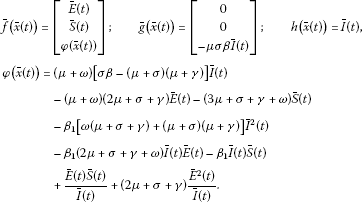(3.5)

The equations in (3.3) define a mapping $\mathrm{\Phi }:{\left[I\phantom{\rule{0.25em}{0ex}}E\phantom{\rule{0.25em}{0ex}}S\right]}^{T}\to {\left[\overline{I}\phantom{\rule{0.25em}{0ex}}\overline{E}\phantom{\rule{0.25em}{0ex}}\overline{S}\right]}^{T}$ whose Jacobian matrix $J\left(x\left(t\right)\right)\stackrel{\mathrm{\Delta }}{=}\left[{J}_{i,j}\left(x\left(t\right)\right)\right]\in {\mathbb{R}}^{3×3}$, with ${J}_{i,j}\left(x\left(t\right)\right)\stackrel{\mathrm{\Delta }}{=}\left[\frac{\partial {\overline{x}}_{i}\left(t\right)}{\partial {x}_{j}\left(t\right)}\right]$ for $i,j\in \left\{1,2,3\right\}$, is non-singular $\mathrm{\forall }x\in D$ since $Det\left[J\left(x\left(t\right)\right)\right]={\sigma }^{2}{\beta }_{1}I\left(t\right)\ne 0$ if $I\left(t\right)\ne 0$. Then the reverse transformation, namely ${\mathrm{\Phi }}^{-1}:{\left[\overline{I}\phantom{\rule{0.25em}{0ex}}\overline{E}\phantom{\rule{0.25em}{0ex}}\overline{S}\right]}^{T}\to {\left[I\phantom{\rule{0.25em}{0ex}}E\phantom{\rule{0.25em}{0ex}}S\right]}^{T}$, is available in order to obtain the original state vector $x\left(t\right)$ from the new one $\overline{x}\left(t\right)$ whenever $I=\overline{I}\ne 0$. By direct calculations, such a reverse transformation is given by

$\begin{array}{r}I\left(t\right)=\overline{I}\left(t\right);\phantom{\rule{2em}{0ex}}E\left(t\right)=\frac{1}{\sigma }\left[\left(\mu +\gamma \right)\overline{I}\left(t\right)+\overline{E}\left(t\right)\right];\\ S\left(t\right)=\frac{1}{\sigma {\beta }_{1}\overline{I}\left(t\right)}\left[\left(\mu +\gamma \right)\left(\mu +\sigma \right)\overline{I}\left(t\right)+\left(2\mu +\sigma +\gamma \right)\overline{E}\left(t\right)+\overline{S}\left(t\right)\right].\end{array}$
(3.6)

Both transformations $\mathrm{\Phi }\left(x\left(t\right)\right)$ and ${\mathrm{\Phi }}^{-1}\left(\overline{x}\left(t\right)\right)$ are smooth mappings, i.e. they have continuous partial derivatives of any order. Then $\mathrm{\Phi }\left(x\left(t\right)\right)$ defines a diffeomorphism on D. The feature that the relative degree of the system is equal to the system order $\mathrm{\forall }x\in D$ allows to change it into a linear and controllable one around any point $x\in D$ via the coordinate transformation (3.3) and an exact linearization feedback control [23, 28]. The following result being relative to the input-output linearization of the system is established.

Theorem 3.1 The state feedback control law defined as

$u\left(t\right)=\frac{1}{{L}_{g}{L}_{f}^{2}h\left(x\left(t\right)\right)}\left[-{L}_{f}^{3}h\left(x\left(t\right)\right)-{\lambda }_{0}h\left(x\left(t\right)\right)-{\lambda }_{1}{L}_{f}h\left(x\left(t\right)\right)-{\lambda }_{2}{L}_{f}^{2}h\left(x\left(t\right)\right)\right],$
(3.7)

where ${\lambda }_{i}$ for $i\in \left\{0,1,2\right\}$ are the controller tuning parameters, induces the linear closed-loop dynamics given by

$\stackrel{⃛}{y}\left(t\right)+{\lambda }_{2}\stackrel{¨}{y}\left(t\right)+{\lambda }_{1}\stackrel{˙}{y}\left(t\right)+{\lambda }_{0}y\left(t\right)=0$
(3.8)

around any point $x\in D$.

Proof The following state equation for the closed-loop system is obtained:

$\left[\begin{array}{c}\stackrel{˙}{\overline{I}}\left(t\right)\\ \stackrel{˙}{\overline{E}}\left(t\right)\\ \stackrel{˙}{\overline{S}}\left(t\right)\end{array}\right]=\left[\begin{array}{c}\overline{E}\left(t\right)\\ \overline{S}\left(t\right)\\ \phi \left(\overline{x}\left(t\right)\right)-{L}_{f}^{3}h\left(x\left(t\right)\right)-{\lambda }_{0}\overline{I}\left(t\right)-{\lambda }_{1}\overline{E}\left(t\right)-{\lambda }_{2}\overline{S}\left(t\right)\end{array}\right]$
(3.9)

by introducing the control law (3.7) in (3.4) and taking into account the coordinate transformation (3.3) and the fact that ${L}_{g}{L}_{f}^{2}h\left(x\left(t\right)\right)=-\mu \sigma \beta I\left(t\right)=-\mu \sigma \beta \overline{I}\left(t\right)\ne 0$ $\mathrm{\forall }x\in D$. Moreover, it follows by direct calculations that

$\begin{array}{rcl}{L}_{f}^{3}h\left(x\left(t\right)\right)& =& \left[\sigma \beta \left(\mu +\omega \right)-{\left(\mu +\gamma \right)}^{3}\right]I\left(t\right)+\sigma \left[{\left(\mu +\gamma \right)}^{2}+\left(2\mu +\sigma +\gamma \right)\left(\mu +\sigma \right)\right]E\left(t\right)\\ -\sigma {\beta }_{1}\omega I\left(t\right)\left[I\left(t\right)+E\left(t\right)\right]\\ -\sigma {\beta }_{1}\left(4\mu +\sigma +2\gamma +\omega \right)I\left(t\right)S\left(t\right)+{\sigma }^{2}{\beta }_{1}E\left(t\right)S\left(t\right)-\sigma {\beta }_{1}^{2}{I}^{2}\left(t\right)S\left(t\right).\end{array}$
(3.10)

One may express ${L}_{f}^{3}h\left(x\left(t\right)\right)$ in the state space defined by $\overline{x}\left(t\right)$ via the application of the coordinate transformation in (3.6). Then it follows directly that ${L}_{f}^{3}h\left(x\left(t\right)\right)=\phi \left(\overline{x}\left(t\right)\right)$. Thus, the state equation of the closed-loop system in the state space defined by $\overline{x}\left(t\right)$ can be written as

(3.11)

Furthermore, the output equation of the closed-loop system is $y\left(t\right)=C\overline{x}\left(t\right)$ with $C=\left[1\phantom{\rule{0.25em}{0ex}}0\phantom{\rule{0.25em}{0ex}}0\right]$ since $y\left(t\right)=I\left(t\right)=\overline{I}\left(t\right)$. From (3.11) and the closed-loop output equation, it follows that

(3.12)

with denoting the order of the differentiation of $y\left(t\right)$. Finally, the dynamics of the closed-loop system (3.8) is obtained by direct calculations from (3.12). □

Remarks 3.1 (i) The controller parameters ${\lambda }_{i}$, for $i\in \left\{0,1,2\right\}$, will be adjusted so that the roots of the closed-loop system characteristic polynomial $P\left(s\right)=Det\left(s{I}_{3}-A\right)$ are located at prescribed positions, i.e. ${\lambda }_{i}={\lambda }_{i}\left(-{r}_{j}\right)$ for $i\in \left\{0,1,2\right\}$ and $j\in \left\{1,\mathrm{2},\mathrm{3}\right\}$, with $\left(-{r}_{j}\right)$ denoting the desired roots of $P\left(s\right)$. If one of the control objectives is to guarantee the exponential stability of the closed-loop system, then all roots of $P\left(s\right)=\left(s+{r}_{1}\right)\left(s+{r}_{2}\right)\left(s+{r}_{3}\right)$ have to be in the open left-half plane, i.e. $Re\left\{{r}_{j}\right\}>0$ for all $j\in \left\{1,2,3\right\}$. Then the values ${\lambda }_{0}={r}_{1}{r}_{2}{r}_{3}>0$, ${\lambda }_{1}={r}_{1}{r}_{2}+{r}_{1}{r}_{3}+{r}_{2}{r}_{3}>0$ and ${\lambda }_{2}={r}_{1}+{r}_{2}+{r}_{3}>0$ for the controller parameters have to be chosen in order to achieve such a stability result. It implies that the strict positivity of the controller parameters is a necessary condition for the exponential stability of the closed-loop system.

1. (ii)

The control (3.7) may be rewritten as follows:

$\begin{array}{rcl}u\left(t\right)& =& \frac{\left(\mu +\omega \right)\sigma \beta -{\left(\mu +\gamma \right)}^{3}+{\lambda }_{0}-{\lambda }_{1}\left(\mu +\gamma \right)+{\lambda }_{2}{\left(\mu +\gamma \right)}^{2}}{\mu \sigma \beta }\\ -\frac{\omega }{\mu N}\left[I\left(t\right)+E\left(t\right)\right]-\frac{\left(3\mu +\sigma +2\gamma -{\lambda }_{2}\right)}{\mu N}S\left(t\right)\\ +\frac{{\left(\mu +\gamma \right)}^{2}+\left(2\mu +\sigma +\gamma \right)\left(\mu +\sigma \right)+{\lambda }_{1}-{\lambda }_{2}\left(2\mu +\sigma +\gamma \right)}{\mu \beta }\frac{E\left(t\right)}{I\left(t\right)}\\ +\frac{\sigma }{\mu N}\frac{E\left(t\right)S\left(t\right)}{I\left(t\right)}-\frac{\beta }{\mu {N}^{2}}I\left(t\right)S\left(t\right)\end{array}$
(3.13)

by using (3.3) and (3.10), or

$\begin{array}{rcl}u\left(t\right)& =& \frac{1}{\mu \sigma \beta \overline{I}\left(t\right)}\left[\phi \left(\overline{x}\left(t\right)\right)+{\lambda }_{0}\overline{I}\left(t\right)+{\lambda }_{1}\overline{E}\left(t\right)+{\lambda }_{2}\overline{S}\left(t\right)\right]\\ =& \frac{1}{\mu \sigma \beta \overline{I}\left(t\right)}\left[\phi \left(\overline{x}\left(t\right)\right)+{\mathrm{\Lambda }}^{T}\overline{x}\left(t\right)\right],\end{array}$
(3.14)

where $\mathrm{\Lambda }\stackrel{\mathrm{\Delta }}{=}{\left[{\lambda }_{0}\phantom{\rule{0.25em}{0ex}}{\lambda }_{1}\phantom{\rule{0.25em}{0ex}}{\lambda }_{2}\right]}^{T}$ is the control parameters vector, by using (3.3) and the facts that ${L}_{f}^{3}h\left(x\left(t\right)\right)=\phi \left(\overline{x}\left(t\right)\right)$ and ${L}_{g}{L}_{f}^{2}h\left(x\left(t\right)\right)=-\mu \sigma \beta \overline{I}\left(t\right)$.

1. (iii)

The control law (3.7) is well defined for all $x\in {\mathbb{R}}_{0+}^{3}$ except in the surface $I=0$. However, the infection may be considered eradicated from the population once the infectious population strictly exceeds zero while it is smaller than one individual. So the vaccination strategy may be switched off when $0<{\delta }^{\prime }\le I\left(t\right)\le \delta <1$. This fact implies that the singularity in the control law is not going to be reached, i.e. such a control law is well defined by the nature of the system. In this sense, the control law given by

(3.15)

may be used instead of (3.7) in a practical situation. The signal $u\left(t\right)$ in (3.15) is given by the linearizing control law (3.7) while ${t}_{f}$ denotes the eventual time instant after which the infection propagation may be assumed ended. Formally, such a time instant is defined as follows:

(3.16)

In this way, the control action is maintained active while the infection persists within the host population and it is switched off once the epidemics is eradicated.

1. (iv)

The linear system (3.8) is strictly identical to the SEIR model (2.1)-(2.4) under the transformation (3.3) and the control law (3.15) for $0\le t\le {t}_{f}$, i.e. until the time instant at which the epidemics is eradicated.

2. (v)

The implementation of the control law (3.15) requires online measurement of the susceptible, infected and infectious population. In a practical situation, only online measures of the infectious and whole populations may be feasible, so the populations of susceptible and infected can only be estimated. In this context, a complete state observer is going to be designed for such a purpose in Section 4.

### 3.1 Controller tuning parameters choice

The application of the control law (3.7), obtained from the exact input-output linearization strategy, makes the closed-loop dynamics of the infectious population be given by (3.8). Such a dynamics depends on the control parameters ${\lambda }_{i}$ for $i\in \left\{0,1,2\right\}$. Such parameters have to be appropriately chosen in order to guarantee the following suitable properties: (i) the stability of the controlled SEIR model, (ii) the eradication of the infection, i.e. the asymptotic convergence of $I\left(t\right)$ and $E\left(t\right)$ to zero as time tends to infinity and (iii) the positivity property of the controlled SEIR model under a vaccination based on such a control strategy. The following theorems related to the choice of the controller tuning parameter values ${\lambda }_{i}$ for $i\in \left\{0,1,2\right\}$ are proven, in order to meet such properties under an eventual vaccination effort.

Theorem 3.2 Assume that the initial condition $x\left(0\right)={\left[I\left(0\right)\phantom{\rule{0.25em}{0ex}}E\left(0\right)\phantom{\rule{0.25em}{0ex}}S\left(0\right)\right]}^{T}\in {\mathbb{R}}_{0+}^{3}$ is bounded, and all roots $\left(-{r}_{j}\right)$ for $j\in \left\{1,2,3\right\}$ of the characteristic polynomial $P\left(s\right)$ associated with the closed-loop dynamics (3.8) are of strictly negative real part via an appropriate choice of the free-design controller parameters ${\lambda }_{i}>0$ for $i\in \left\{0,1,2\right\}$. Then the control law (3.7) guarantees the exponential stability of the transformed controlled SEIR model (3.1)-(3.6) while achieving the eradication of the infection from the host population as time tends to infinity. Moreover, the SEIR model (2.1)-(2.4) has the following properties: $E\left(t\right)$, $I\left(t\right)$, $S\left(t\right)I\left(t\right)$ and $S\left(t\right)+R\left(t\right)=N-\left[E\left(t\right)+I\left(t\right)\right]$ are bounded for all time, $E\left(t\right)\to 0$, $I\left(t\right)\to 0$, $S\left(t\right)+R\left(t\right)\to N$ and $S\left(t\right)I\left(t\right)\to 0$ exponentially as $t\to \mathrm{\infty }$, and $I\left(t\right)=o\left(1/S\left(t\right)\right)$.

Proof The dynamics of the controlled SEIR model (3.8) can be equivalently rewritten with the state equation (3.11) and the output equation $y\left(t\right)=C\overline{x}\left(t\right)$, where $C=\left[1\phantom{\rule{0.25em}{0ex}}0\phantom{\rule{0.25em}{0ex}}0\right]$, by taking into account that $y\left(t\right)=\overline{I}\left(t\right)$, $\stackrel{˙}{y}\left(t\right)=\overline{E}\left(t\right)$ and $\stackrel{¨}{y}\left(t\right)=\overline{S}\left(t\right)$. The initial condition $\overline{x}\left(0\right)={\left[\overline{I}\left(0\right)\phantom{\rule{0.25em}{0ex}}\overline{E}\left(0\right)\phantom{\rule{0.25em}{0ex}}\overline{S}\left(0\right)\right]}^{T}$ in such a realization is bounded since it is related to $x\left(0\right)$ via the coordinate transformation (3.3), and $x\left(0\right)$ is assumed to be bounded. The controlled SEIR model is exponentially stable since the eigenvalues of the matrix A are the roots $\left(-{r}_{j}\right)$ for $j\in \left\{1,2,3\right\}$ of $P\left(s\right)$ which are assumed to be in the open left-half plane. Then the state vector $\overline{x}\left(t\right)$ exponentially converges to zero as time tends to infinity while being bounded for all time. Moreover, $I\left(t\right)$ and $E\left(t\right)$ are also bounded and converge exponentially to zero as $t\to \mathrm{\infty }$ from the boundedness and exponential convergence to zero of $\overline{x}\left(t\right)$ as $t\to \mathrm{\infty }$ according to the first and second equations of the coordinate transformation (3.3). Then the infection is eradicated from the host population. Furthermore, the boundedness of $S\left(t\right)+R\left(t\right)$ follows from that of $E\left(t\right)$ and $I\left(t\right)$, and the fact that the total population is constant for all time. Also, the exponential convergence of $S\left(t\right)+R\left(t\right)$ to the total population as $t\to \mathrm{\infty }$ is derived from the exponential convergence to zero of $I\left(t\right)$ and $E\left(t\right)$ as $t\to \mathrm{\infty }$, and the fact that $S\left(t\right)+E\left(t\right)+I\left(t\right)+R\left(t\right)=N$ $\mathrm{\forall }t\in {\mathbb{R}}_{0+}$. Finally, from the third equation of (3.3), it follows that $S\left(t\right)I\left(t\right)$ is bounded and it converges exponentially to zero as $t\to \mathrm{\infty }$ from the boundedness and convergence to zero of $I\left(t\right)$, $E\left(t\right)$ and $\overline{x}\left(t\right)$ as $t\to \mathrm{\infty }$. The facts that $I\left(t\right)\to 0$ and $S\left(t\right)I\left(t\right)\to 0$ as $t\to \mathrm{\infty }$ imply directly that $I\left(t\right)=o\left(1/S\left(t\right)\right)$. □

Remark 3.2 Theorem 3.2 implies the existence of a finite time instant ${t}_{f}$ after which the epidemics is eradicated if the vaccination control law (3.15) is used instead of that in (3.7). Concretely, such an existence derives from the convergence of $I\left(t\right)$ to zero as $t\to \mathrm{\infty }$ via the application of the control law (3.7).

Theorem 3.3 Assume that an initial condition for the SEIR model satisfies $R\left(0\right)\ge 0$, $x\left(0\right)\in {\mathbb{R}}_{0+}^{3}$, i.e. $I\left(0\right)\ge 0$, $E\left(0\right)\ge 0$ and $S\left(0\right)\ge 0$, and the constraint $S\left(0\right)+E\left(0\right)+I\left(0\right)+R\left(0\right)=N$. Assume also that some strictly positive real numbers ${r}_{j}$ for $j\in \left\{1,2,3\right\}$ are chosen such that

1. (a)

$0<{r}_{1}<\mu +min\left\{\sigma ,\gamma \right\}$, ${r}_{2}=\mu +\gamma$ and ${r}_{3}>\mu +max\left\{\sigma ,\gamma \right\}$, so that ${r}_{3}>{r}_{2}>{r}_{1}>0$,

2. (b)

${r}_{1}$ and ${r}_{3}$ satisfy the inequalities:

$\left\{\begin{array}{c}{r}_{1}+{r}_{3}\ge 2\mu +\sigma +\gamma +\beta -\omega ,\hfill \\ {r}_{1}{r}_{3}\ge \left(\mu +\sigma \right)\left({r}_{1}+{r}_{3}\right)+\left(\gamma -\sigma \right)\left(2\mu +\sigma +\gamma \right)-{\left(\mu +\gamma \right)}^{2},\hfill \\ \left({r}_{3}-{r}_{1}\right)\left({r}_{3}-\mu -\gamma \right)\ge \sigma \beta .\hfill \end{array}$

Then

1. (i)

the application of the control law (3.7) to the SEIR model guarantees that the epidemics is asymptotically eradicated from the host population while $I\left(t\right)\ge 0$, $E\left(t\right)\ge 0$ and $S\left(t\right)\ge 0$ $\mathrm{\forall }t\in {\mathbb{R}}_{0+}$, and

2. (ii)

the application of the control law (3.15) guarantees the epidemics eradication after a finite time ${t}_{f}$, the positivity of the controlled SEIR epidemic model $\mathrm{\forall }t\in {\mathbb{R}}_{0}^{+}$ and that $u\left(t\right)=V\left(t\right)\ge 1$ $\mathrm{\forall }t\in \left[0,{t}_{f}\right)$ so that $u\left(t\right)\ge 0$ $\mathrm{\forall }t\in {\mathbb{R}}_{0+}$,

provided that the controller tuning parameters ${\lambda }_{i}$ for $i\in \left\{0,1,2\right\}$ are chosen such that $\left(-{r}_{j}\right)$ for $j\in \left\{1,2,3\right\}$ are the roots of the characteristic polynomial $P\left(s\right)$ associated with the closed loop dynamics (3.8).

Proof (i) On the one hand, the epidemics asymptotic eradication is proven by following the same reasoning as in Theorem 3.2. On the other hand, the dynamics of the controlled SEIR model (3.8) can be written in the state space defined by $\overline{x}\left(t\right)={\left[\overline{I}\left(t\right)\phantom{\rule{0.25em}{0ex}}\overline{E}\left(t\right)\phantom{\rule{0.25em}{0ex}}\overline{S}\left(t\right)\right]}^{T}$ as in (3.11). From such a realization, taking into account the first equation in (3.3) and the fact that $\left(-{r}_{j}\right)$ for $j\in \left\{1,2,3\right\}$ are the eigenvalues of A, it follows that

$I\left(t\right)=\overline{I}\left(t\right)=y\left(t\right)={c}_{1}{e}^{-{r}_{1}t}+{c}_{2}{e}^{-{r}_{2}t}+{c}_{3}{e}^{-{r}_{3}t}\phantom{\rule{1em}{0ex}}\mathrm{\forall }t\in {\mathbb{R}}_{0+}$
(3.17)

for some constants ${c}_{j}$ for $j\in \left\{1,2,3\right\}$ being dependent on the initial conditions $y\left(0\right)$, $\stackrel{˙}{y}\left(0\right)$ and $\stackrel{¨}{y}\left(0\right)$. In turn, such initial conditions are related to the initial conditions of the SEIR model in its original realization, i.e. in the state space defined by $x\left(t\right)={\left[I\left(t\right)\phantom{\rule{0.25em}{0ex}}E\left(t\right)\phantom{\rule{0.25em}{0ex}}S\left(t\right)\right]}^{T}$ via (3.3). The constants ${c}_{j}$ for $j\in \left\{1,2,3\right\}$ can be obtained by solving the following set of linear equations:

$\begin{array}{r}\overline{I}\left(0\right)=y\left(0\right)={c}_{1}+{c}_{2}+{c}_{3}=I\left(0\right),\\ \overline{E}\left(0\right)=\stackrel{˙}{y}\left(0\right)=-\left({c}_{1}{r}_{1}+{c}_{2}{r}_{2}+{c}_{3}{r}_{3}\right)=-\left(\mu +\gamma \right)I\left(0\right)+\sigma E\left(0\right),\\ \overline{S}\left(0\right)=\stackrel{¨}{y}\left(0\right)={c}_{1}{r}_{1}^{2}+{c}_{2}{r}_{2}^{2}+{c}_{3}{r}_{3}^{2}\\ \phantom{\overline{S}\left(0\right)}={\left(\mu +\gamma \right)}^{2}I\left(0\right)-\sigma \left(2\mu +\sigma +\gamma \right)E\left(0\right)+\sigma {\beta }_{1}I\left(0\right)S\left(0\right),\end{array}$
(3.18)

where (3.3) and (3.17) have been used. Such equations can be more compactly written as ${R}_{p}\cdot K=M$, where

$\begin{array}{r}{R}_{p}=\left[\begin{array}{ccc}1& 1& 1\\ {r}_{1}& {r}_{2}& {r}_{3}\\ {r}_{1}^{2}& {r}_{2}^{2}& {r}_{3}^{2}\end{array}\right],\phantom{\rule{2em}{0ex}}K=\left[\begin{array}{c}{c}_{1}\\ {c}_{2}\\ {c}_{3}\end{array}\right]\phantom{\rule{1em}{0ex}}\text{and}\\ M=\left[\begin{array}{c}I\left(0\right)\\ \left(\mu +\gamma \right)I\left(0\right)-\sigma E\left(0\right)\\ {\left(\mu +\gamma \right)}^{2}I\left(0\right)-\sigma \left(2\mu +\sigma +\gamma \right)E\left(0\right)+\sigma {\beta }_{1}I\left(0\right)S\left(0\right)\end{array}\right].\end{array}$
(3.19)

Once the desired roots of the characteristic equation of the closed-loop dynamics have been prefixed, the constants ${c}_{j}$ for $j\in \left\{1,2,3\right\}$ of the time-evolution of $I\left(t\right)$ are obtained from $K={R}_{p}^{-1}M$ since ${R}_{p}$ is a non-singular matrix, i.e. an invertible matrix. In this sense, note that $Det\left({R}_{p}\right)=\left({r}_{2}-{r}_{1}\right)\left({r}_{3}-{r}_{1}\right)\left({r}_{3}-{r}_{2}\right)\ne 0$ since ${R}_{p}$ is the Vandermonde matrix  and the roots $\left(-{r}_{j}\right)$ for $j\in \left\{1,2,3\right\}$ have been chosen different among them. Namely

$K=\left[\begin{array}{c}{c}_{1}\\ {c}_{2}\\ {c}_{3}\end{array}\right]=\left[\begin{array}{c}\frac{F\left({r}_{2},{r}_{3}\right)I\left(0\right)+\sigma G\left({r}_{2},{r}_{3}\right)E\left(0\right)+\sigma {\beta }_{1}I\left(0\right)S\left(0\right)}{\left({r}_{2}-{r}_{1}\right)\left({r}_{3}-{r}_{1}\right)}\\ -\frac{F\left({r}_{1},{r}_{3}\right)I\left(0\right)+\sigma G\left({r}_{1},{r}_{3}\right)E\left(0\right)+\sigma {\beta }_{1}I\left(0\right)S\left(0\right)}{\left({r}_{2}-{r}_{1}\right)\left({r}_{3}-{r}_{2}\right)}\\ \frac{F\left({r}_{1},{r}_{2}\right)I\left(0\right)+\sigma G\left({r}_{1},{r}_{2}\right)E\left(0\right)+\sigma {\beta }_{1}I\left(0\right)S\left(0\right)}{\left({r}_{3}-{r}_{1}\right)\left({r}_{3}-{r}_{2}\right)}\end{array}\right],$
(3.20)

where the functions $F:{\mathbb{R}}_{+}^{2}\to \mathbb{R}$ and $G:{\mathbb{R}}_{+}^{2}\to \mathbb{R}$ are defined as follows:

$\begin{array}{r}F\left(v,w\right)=vw-\left(\mu +\gamma \right)\left(v+w\right)+{\left(\mu +\gamma \right)}^{2}\phantom{\rule{1em}{0ex}}\text{and}\\ G\left(v,w\right)=v+w-\left(2\mu +\sigma +\gamma \right).\end{array}$
(3.21)

In particular, ${c}_{1}=\frac{\sigma \left({r}_{3}-\mu -\gamma \right)E\left(0\right)+\sigma {\beta }_{1}I\left(0\right)S\left(0\right)}{\left(\mu +\gamma -{r}_{1}\right)\left({r}_{3}-{r}_{1}\right)}>0$ since $I\left(0\right)\ge 0$, $S\left(0\right)\ge 0$, $E\left(0\right)\ge 0$, $F\left({r}_{2},{r}_{3}\right)=0$, $G\left({r}_{2},{r}_{3}\right)={r}_{3}-\mu -\gamma >0$, $\mu +\gamma -{r}_{1}>0$ and ${r}_{3}-{r}_{1}>0$ by taking into account the constraints in (a). On the one hand, $I\left(t\right)\ge 0$ $\mathrm{\forall }t\in {\mathbb{R}}_{0+}$ is proven directly from (3.17) as follows. One ‘a priori’ knows that ${c}_{1}>0$. However, the sign of both ${c}_{2}$ and ${c}_{3}$ may not be ‘a priori’ determined from the initial conditions and constraints in (a). The following four cases may be possible: (i) ${c}_{2}\ge 0$ and ${c}_{3}\ge 0$, (ii) ${c}_{2}\ge 0$ and ${c}_{3}<0$, (iii) ${c}_{2}<0$ and ${c}_{3}\ge 0$, and (iv) ${c}_{2}<0$ and ${c}_{3}<0$. For the cases (i) and (ii), i.e. if ${c}_{2}\ge 0$, it follows from (3.17) that

$\begin{array}{rcl}I\left(t\right)& =& {c}_{1}{e}^{-{r}_{1}t}+{c}_{2}{e}^{-{r}_{2}t}+\left[I\left(0\right)-{c}_{1}-{c}_{2}\right]{e}^{-{r}_{3}t}\\ =& {c}_{1}\left({e}^{-{r}_{1}t}-{e}^{-{r}_{3}t}\right)+{c}_{2}\left({e}^{-{r}_{2}t}-{e}^{-{r}_{3}t}\right)+I\left(0\right){e}^{-{r}_{3}t}\ge 0\phantom{\rule{1em}{0ex}}\mathrm{\forall }t\in {\mathbb{R}}_{0+},\end{array}$
(3.22)

where the facts that $I\left(0\right)={c}_{1}+{c}_{2}+{c}_{3}\ge 0$ and, ${e}^{-{r}_{1}t}-{e}^{-{r}_{3}t}\ge 0$ and ${e}^{-{r}_{2}t}-{e}^{-{r}_{3}t}\ge 0$ $\mathrm{\forall }t\in {\mathbb{R}}_{0+}$ since ${r}_{1}<{r}_{2}<{r}_{3}$, have been taken into account. For the case (iii), i.e. if ${c}_{2}<0$ and ${c}_{3}\ge 0$, it follows from (3.17) that

$\begin{array}{rcl}I\left(t\right)& =& \left[I\left(0\right)-{c}_{2}-{c}_{3}\right]{e}^{-{r}_{1}t}+{c}_{2}{e}^{-{r}_{2}t}+{c}_{3}{e}^{-{r}_{3}t}\\ =& \left[I\left(0\right)-{c}_{3}\right]{e}^{-{r}_{1}t}+{c}_{2}\left({e}^{-{r}_{2}t}-{e}^{-{r}_{1}t}\right)+{c}_{3}{e}^{-{r}_{3}t}\ge 0\phantom{\rule{1em}{0ex}}\mathrm{\forall }t\in {\mathbb{R}}_{0+},\end{array}$
(3.23)

by taking into account that $I\left(0\right)={c}_{1}+{c}_{2}+{c}_{3}$, ${e}^{-{r}_{2}t}-{e}^{-{r}_{1}t}\le 0$ $\mathrm{\forall }t\in {\mathbb{R}}_{0+}$ since ${r}_{1}<{r}_{2}$ and the fact that

$I\left(0\right)-{c}_{3}=\frac{\left[\left({r}_{3}-{r}_{1}\right)\left({r}_{3}-\mu -\gamma \right)-\sigma {\beta }_{1}S\left(0\right)\right]I\left(0\right)+\sigma \left(\mu +\gamma -{r}_{1}\right)E\left(0\right)}{\left({r}_{3}-{r}_{1}\right)\left({r}_{3}-\mu -\gamma \right)}\ge 0,$
(3.24)

where (3.20), (3.21), $F\left({r}_{1},{r}_{2}\right)=0$, $G\left({r}_{1},{r}_{2}\right)={r}_{1}-\mu -\gamma <0$ and the constraints in (a) and (b) have been used. In particular, the coefficient multiplying to $I\left(0\right)$ in (3.24) is non-negative if ${r}_{1}$ and ${r}_{3}$ satisfy the third inequality of the constraints (b) by taking into account $\sigma {\beta }_{1}S\left(0\right)=\sigma \beta \frac{S\left(0\right)}{N}\le \sigma \beta$ and $S\left(0\right)\le N$. This later inequality is directly implied by $I\left(0\right)\ge 0$, $E\left(0\right)\ge 0$, $S\left(0\right)\ge 0$, $R\left(0\right)\ge 0$ and $N=I\left(0\right)+E\left(0\right)+S\left(0\right)+R\left(0\right)$. Finally, for the case (iv), i.e. if ${c}_{2}<0$ and ${c}_{3}<0$, it follows from (3.17) that

$\begin{array}{rcl}I\left(t\right)& =& \left[I\left(0\right)-{c}_{2}-{c}_{3}\right]{e}^{-{r}_{1}t}+{c}_{2}{e}^{-{r}_{2}t}+{c}_{3}{e}^{-{r}_{3}t}\\ =& I\left(0\right){e}^{-{r}_{1}t}+{c}_{2}\left({e}^{-{r}_{2}t}-{e}^{-{r}_{1}t}\right)+{c}_{3}\left({e}^{-{r}_{3}t}-{e}^{-{r}_{1}t}\right)\ge 0\phantom{\rule{1em}{0ex}}\mathrm{\forall }t\in {\mathbb{R}}_{0+},\end{array}$
(3.25)

where the constraints $I\left(0\right)={c}_{1}+{c}_{2}+{c}_{3}\ge 0$, ${e}^{-{r}_{2}t}-{e}^{-{r}_{1}t}\le 0$ and ${e}^{-{r}_{3}t}-{e}^{-{r}_{1}t}\le 0$ $\mathrm{\forall }t\in {\mathbb{R}}_{0+}$, since ${r}_{1}<{r}_{2}<{r}_{3}$, have been taken into account. In summary, $I\left(t\right)\ge 0$ $\mathrm{\forall }t\in {\mathbb{R}}_{0+}$ if all partial populations are initially non-negative and the roots $\left(-{r}_{j}\right)$ for $j\in \left\{1,2,3\right\}$of the closed-loop characteristic polynomial satisfy the constraints in (a) and (b). On the other hand, one obtains by direct calculations from (3.6) and (3.17) that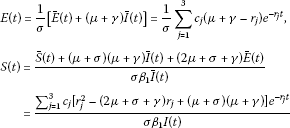(3.26)

by taking into account that $\overline{E}\left(t\right)=\stackrel{˙}{\overline{I}}\left(t\right)$ and $\overline{S}\left(t\right)=\stackrel{¨}{\overline{I}}\left(t\right)$. If one fixes the parameter ${r}_{2}=\mu +\gamma$ then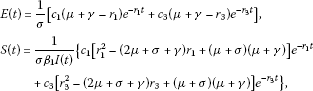(3.27)

where the fact that the function $H:{\mathbb{R}}_{+}\to \mathbb{R}$ defined by

$H\left(v\right)={v}^{2}-\left(2\mu +\sigma +\gamma \right)v+\left(\mu +\sigma \right)\left(\mu +\gamma \right)$
(3.28)

is zero for $v={r}_{2}=\mu +\gamma$ has been used. From the first equation in (3.27), it follows that ${c}_{3}\left(\mu +\gamma -{r}_{3}\right)=\sigma E\left(0\right)-{c}_{1}\left(\mu +\gamma -{r}_{1}\right)$ and then

$E\left(t\right)=\frac{1}{\sigma }\left[{c}_{1}\left(\mu +\gamma -{r}_{1}\right)\left({e}^{-{r}_{1}t}-{e}^{-{r}_{3}t}\right)+\sigma E\left(0\right){e}^{-{r}_{3}t}\right]\ge 0\phantom{\rule{1em}{0ex}}\mathrm{\forall }t\in {\mathbb{R}}_{0+}$
(3.29)

by applying such a relation between ${c}_{1}$ and ${c}_{3}$ in (3.27) and by taking into account that ${c}_{1}\left(\mu +\gamma -{r}_{1}\right)>0$, $E\left(0\right)\ge 0$ and ${e}^{-{r}_{1}t}-{e}^{-{r}_{3}t}\ge 0$ $\mathrm{\forall }t\in {\mathbb{R}}_{0+}$ since ${r}_{1}<{r}_{3}$. In this way, the non-negativity of $E\left(t\right)$ has been proven. From the second equation in (3.27), it follows that ${c}_{3}H\left({r}_{3}\right)=\sigma {\beta }_{1}I\left(0\right)S\left(0\right)-{c}_{1}H\left({r}_{1}\right)$ and then

$S\left(t\right)=\frac{1}{\sigma {\beta }_{1}I\left(t\right)}\left[{c}_{1}H\left({r}_{1}\right)\left({e}^{-{r}_{1}t}-{e}^{-{r}_{3}t}\right)+\sigma {\beta }_{1}I\left(0\right)S\left(0\right){e}^{-{r}_{3}t}\right]\ge 0\phantom{\rule{1em}{0ex}}\mathrm{\forall }t\in {\mathbb{R}}_{0+}$
(3.30)

by applying such a relation between ${c}_{1}$ and ${c}_{3}$ in (3.27) and by taking into account that ${c}_{1}H\left({r}_{1}\right)>0$ since ${r}_{1}<\mu +min\left\{\sigma ,\gamma \right\}$, $I\left(0\right)\ge 0$, $S\left(0\right)\ge 0$, and $I\left(t\right)\ge 0$ and ${e}^{-{r}_{1}t}-{e}^{-{r}_{3}t}\ge 0$ $\mathrm{\forall }t\in {\mathbb{R}}_{0+}$ since ${r}_{1}<{r}_{3}$. In this way, the non-negativity of $S\left(t\right)$ has been proven. Note that the function $H\left(v\right)$ defined by (3.28) is an upper-open parabola zero-valued for ${v}_{1}=\mu +\sigma$ and ${v}_{2}=\mu +\gamma$ so $H\left({r}_{1}\right)>0$ from the assumption that ${r}_{1}<\mu +min\left\{\sigma ,\gamma \right\}$.

1. (ii)

On the one hand, if the control law (3.15) is used instead of that in (3.7), then the time evolution of the infectious population is also given by (3.17) while the control action is active. Thus, the exponential convergence of $I\left(t\right)$ to zero as $t\to \mathrm{\infty }$ in (3.17) implies directly the existence of a finite time instant ${t}_{f}$ at which the control (3.15) switches off. Obviously, the non-negativity of $I\left(t\right)$, $E\left(t\right)$ and $S\left(t\right)$ $\mathrm{\forall }t\in \left[0,{t}_{f}\right]$ is proven by following the same reasoning used in the part (i) of the current theorem. The non-negativity of $R\left(t\right)$ $\mathrm{\forall }t\in \left[0,{t}_{f}\right]$ is proven by using continuity arguments. In this sense, if $R\left(t\right)$ reaches negative values for some $t\in \left[0,{t}_{f}\right]$ starting from an initial condition $R\left(0\right)\ge 0$, then $R\left(t\right)$ passes through zero, i.e. there exists at least a time instant ${t}_{0}\in \left[0,{t}_{f}\right)$ such that $R\left({t}_{0}\right)=0$. Then it follows from (2.4) that

$\begin{array}{rcl}\stackrel{˙}{R}\left({t}_{0}\right)& =& \gamma I\left({t}_{0}\right)+\mu NV\left({t}_{0}\right)\\ =& \gamma I\left({t}_{0}\right)+\frac{\mu \sigma \beta +{\lambda }_{0}-{\lambda }_{1}\left(\mu +\gamma \right)+{\lambda }_{2}{\left(\mu +\gamma \right)}^{2}-{\left(\mu +\gamma \right)}^{3}}{\sigma \beta }N\\ +\left({\lambda }_{2}+\omega -3\mu -\sigma -2\gamma \right)S\left({t}_{0}\right)\\ +\frac{{\left(\mu +\gamma \right)}^{2}+\left(2\mu +\sigma +\gamma \right)\left(\mu +\sigma \right)+{\lambda }_{1}-{\lambda }_{2}\left(2\mu +\sigma +\gamma \right)}{\beta }N\frac{E\left({t}_{0}\right)}{I\left({t}_{0}\right)}\\ +\sigma \frac{E\left({t}_{0}\right)S\left({t}_{0}\right)}{I\left({t}_{0}\right)}-\frac{\beta }{N}I\left({t}_{0}\right)S\left({t}_{0}\right)\end{array}$
(3.31)

by introducing the control law (3.15) and taking into account the facts that $V\left(t\right)=u\left(t\right)$ and $I\left({t}_{0}\right)+E\left({t}_{0}\right)+S\left({t}_{0}\right)=N$ since $R\left({t}_{0}\right)=0$ has been used. Moreover, the non-negativity of $I\left(t\right)$, $E\left(t\right)$ and $S\left(t\right)$ $\mathrm{\forall }t\in \left[0,{t}_{f}\right]$ as it has been previously proven, implies that $I\left({t}_{0}\right)\le N$, $E\left({t}_{0}\right)\le N$ and $S\left({t}_{0}\right)\le N$. Also, $I\left({t}_{0}\right)\ge \delta >0$ since ${t}_{0}<{t}_{f}$ and from the definition of ${t}_{f}$ in (3.16). Then one obtains

$\begin{array}{rcl}\stackrel{˙}{R}\left({t}_{0}\right)& \ge & \gamma I\left({t}_{0}\right)+\frac{\mu \sigma \beta +{\lambda }_{0}-{\lambda }_{1}\left(\mu +\gamma \right)+{\lambda }_{2}{\left(\mu +\gamma \right)}^{2}-{\left(\mu +\gamma \right)}^{3}}{\sigma \beta }N\\ +\left({\lambda }_{2}+\omega -3\mu -\sigma -2\gamma -\beta \right)S\left({t}_{0}\right)\\ +\frac{{\left(\mu +\gamma \right)}^{2}+\left(2\mu +\sigma +\gamma \right)\left(\mu +\sigma \right)+{\lambda }_{1}-{\lambda }_{2}\left(2\mu +\sigma +\gamma \right)}{\beta }N\frac{E\left({t}_{0}\right)}{I\left({t}_{0}\right)}\\ +\sigma \frac{E\left({t}_{0}\right)S\left({t}_{0}\right)}{I\left({t}_{0}\right)}\end{array}$
(3.32)

from (3.31). The controller tuning parameters ${\lambda }_{i}$ for $i\in \left\{0,1,2\right\}$ are related to the roots $\left(-{r}_{j}\right)$ for $j\in \left\{1,2,3\right\}$ of the closed-loop characteristic polynomial $P\left(s\right)$, see Remark 3.1 (i), by

${\lambda }_{0}={r}_{1}{r}_{2}{r}_{3};\phantom{\rule{2em}{0ex}}{\lambda }_{1}={r}_{1}{r}_{2}+{r}_{1}{r}_{3}+{r}_{2}{r}_{3};\phantom{\rule{2em}{0ex}}{\lambda }_{2}={r}_{1}+{r}_{2}+{r}_{3}.$
(3.33)

The assignment of ${r}_{j}$ for $j\in \left\{1,2,3\right\}$ such that the constraints in (a) and (b) are fulfilled implies that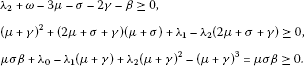(3.34)

Then $\stackrel{˙}{R}\left({t}_{0}\right)\ge 0$ by taking into account (3.34) in (3.32). The facts that $R\left(t\right)\ge 0$ $\mathrm{\forall }t\in \left[0,{t}_{0}\right)$, $R\left({t}_{0}\right)=0$ and $\stackrel{˙}{R}\left({t}_{0}\right)\ge 0$ imply that $R\left(t\right)\ge 0$ $\mathrm{\forall }t\in \left[0,{t}_{f}\right]$ via complete induction. Finally, the positivity of the controlled SEIR model $\mathrm{\forall }t\in {\mathbb{R}}_{0}^{+}$ follows from the non-negativity of $I\left(t\right)$, $E\left(t\right)$, $S\left(t\right)$ and $R\left(t\right)$ $\mathrm{\forall }t\in \left[0,{t}_{f}\right]$ and Lemma 2.1.

On the other hand, it follows from (3.13) and (3.15) that

$\begin{array}{rcl}u\left(t\right)& =& \frac{\mu \sigma \beta -{\left(\mu +\gamma \right)}^{3}+{\lambda }_{0}-{\lambda }_{1}\left(\mu +\gamma \right)+{\lambda }_{2}{\left(\mu +\gamma \right)}^{2}}{\mu \sigma \beta }\\ +\frac{\omega }{\mu N}R\left(t\right)-\frac{\left(3\mu +\sigma +2\gamma -\omega -{\lambda }_{2}\right)}{\mu N}S\left(t\right)\\ +\frac{{\left(\mu +\gamma \right)}^{2}+\left(2\mu +\sigma +\gamma \right)\left(\mu +\sigma \right)+{\lambda }_{1}-{\lambda }_{2}\left(2\mu +\sigma +\gamma \right)}{\mu \beta }\frac{E\left(t\right)}{I\left(t\right)}\\ +\frac{\sigma }{\mu N}\frac{E\left(t\right)S\left(t\right)}{I\left(t\right)}-\frac{\beta }{\mu {N}^{2}}I\left(t\right)S\left(t\right)\end{array}$
(3.35)

$\mathrm{\forall }t\in \left[0,{t}_{f}\right]$ by taking into account that $S\left(t\right)+E\left(t\right)+I\left(t\right)+R\left(t\right)=N$. Moreover,

$\begin{array}{rcl}u\left(t\right)& \ge & \frac{\mu \sigma \beta -{\left(\mu +\gamma \right)}^{3}+{\lambda }_{0}-{\lambda }_{1}\left(\mu +\gamma \right)+{\lambda }_{2}{\left(\mu +\gamma \right)}^{2}}{\mu \sigma \beta }+\frac{{\lambda }_{2}+\omega -3\mu -\sigma -2\gamma -\beta }{\mu N}S\left(t\right)\\ +\frac{{\left(\mu +\gamma \right)}^{2}+\left(2\mu +\sigma +\gamma \right)\left(\mu +\sigma \right)+{\lambda }_{1}-{\lambda }_{2}\left(2\mu +\sigma +\gamma \right)}{\mu \beta }\frac{E\left(t\right)}{I\left(t\right)}\phantom{\rule{1em}{0ex}}\mathrm{\forall }t\in \left[0,{t}_{f}\right],\end{array}$
(3.36)

where the facts that $0<\delta \le I\left(t\right)\le N$, $E\left(t\right)\ge 0$, $S\left(t\right)\ge 0$ and $R\left(t\right)\ge 0$ $\mathrm{\forall }t\in \left[0,{t}_{f}\right]$ have been used. If the roots of the polynomial $P\left(s\right)$ satisfy the conditions in (a) and (b), it follows from (3.36) that

$\begin{array}{rcl}u\left(t\right)& \ge & 1+\frac{{\lambda }_{2}+\omega -3\mu -\sigma -2\gamma -\beta }{\mu N}S\left(t\right)\\ +\frac{{\left(\mu +\gamma \right)}^{2}+\left(2\mu +\sigma +\gamma \right)\left(\mu +\sigma \right)+{\lambda }_{1}-{\lambda }_{2}\left(2\mu +\sigma +\gamma \right)}{\mu \beta }\frac{E\left(t\right)}{I\left(t\right)}\ge 1\end{array}$
(3.37)

$\mathrm{\forall }t\in \left[0,{t}_{f}\right]$ by taking into account the third equation in (3.34) and the non-negativity of $S\left(t\right)$, $E\left(t\right)$ and $I\left(t\right)$ $\mathrm{\forall }t\in \left[0,{t}_{f}\right]$. Finally, it follows that $u\left(t\right)\ge 0$ $\mathrm{\forall }t\in {\mathbb{R}}_{0}^{+}$ from (3.15) and (3.37). □

In summary, this section has dealt with a vaccination strategy based on linearization control techniques for nonlinear systems. The proposed control law satisfies the main objectives required in the field of epidemics models, namely the stability, the positivity and the eradication of the infection from the population. Such results are proven formally in Theorems 3.2 and 3.3. In Section 5, some simulation results illustrate the effectiveness of such a vaccination strategy. However, such a strategy has a main drawback, namely the control law needs the knowledge of the true values of the susceptible, infected and infectious populations at all time instants which are not available in certain real situations. An alternative approach useful to overcome such a drawback is dealt with in the following section where an observer to estimate all the partial populations is proposed.

## 4 Vaccination control strategy based on the use of a state observer

The control laws (3.7), or equivalently (3.13) or (3.14), and (3.15) require the online measurement of all the state variables, namely $I\left(t\right)$, $E\left(t\right)$ and $S\left(t\right)$. However, the online measures of the infected and susceptible populations are rarely affordable in certain real situations where only knowledge about the infectious and total populations may be available. As a consequence, the control laws (3.7) and (3.15) may not be implemented. An alternative approach involving the use of a complete state observer is proposed. This observer provides online estimates $\stackrel{ˆ}{I}\left(t\right)$, $\stackrel{ˆ}{E}\left(t\right)$ and $\stackrel{ˆ}{S}\left(t\right)$ of the true state variables. Such estimates are used instead of $I\left(t\right)$, $E\left(t\right)$ and $S\left(t\right)$ for the implementation of the switching control law given by

$u\left(t\right)=\left\{\begin{array}{cc}0,\hfill & 0\le t<{t}_{1},\hfill \\ {u}_{l}\left(t\right),\hfill & {t}_{1}\le t\le {t}_{f},\hfill \\ 0,\hfill & t>{t}_{f}\hfill \end{array}$
(4.1)

with ${u}_{l}\left(t\right)$ defined as follows:

$\begin{array}{rcl}{u}_{l}\left(t\right)& =& \frac{1}{\mu \sigma \beta \stackrel{ˆ}{\overline{I}}\left(t\right)}\left[\phi \left(\stackrel{ˆ}{\overline{x}}\left(t\right)\right)+{\lambda }_{0}\stackrel{ˆ}{\overline{I}}\left(t\right)+{\lambda }_{1}\stackrel{ˆ}{\overline{E}}\left(t\right)+{\lambda }_{2}\stackrel{ˆ}{\overline{S}}\left(t\right)\right]\\ =& \frac{1}{\mu \sigma \beta \stackrel{ˆ}{\overline{I}}\left(t\right)}\left[\phi \left(\stackrel{ˆ}{\overline{x}}\left(t\right)\right)+{\mathrm{\Lambda }}^{T}\stackrel{ˆ}{\overline{x}}\left(t\right)\right],\end{array}$
(4.2)

where $\stackrel{ˆ}{\overline{x}}\left(t\right)\stackrel{\mathrm{\Delta }}{=}{\left[\stackrel{ˆ}{\overline{I}}\left(t\right)\phantom{\rule{0.25em}{0ex}}\stackrel{ˆ}{\overline{E}}\left(t\right)\phantom{\rule{0.25em}{0ex}}\stackrel{ˆ}{\overline{S}}\left(t\right)\right]}^{T}$ denotes the estimate of the state vector $\overline{x}\left(t\right)$ corresponding to the system representation (3.4)-(3.5). The switching time instants in the control law (4.1), i.e. ${t}_{1}$ and ${t}_{f}$ are defined as follows:

(4.3)

for some real constants ${\delta }_{I}>{\epsilon }_{I}>0$, ${\epsilon }_{u}>0$ and $0<{\delta }_{I}^{\prime }\ll 1$ so that ${\delta }_{I}^{\prime }<{\delta }_{I}-{\epsilon }_{I}$. Note that ${t}_{1}={t}_{1}\left({\epsilon }_{u},{\epsilon }_{I},{\delta }_{I}\right)$ and ${t}_{f}={t}_{f}\left({\delta }_{I}^{\prime }\right)$. The signal ${e}_{I}\left(t\right)\stackrel{\mathrm{\Delta }}{=}\stackrel{ˆ}{I}\left(t\right)-I\left(t\right)$ denotes the estimation error corresponding to the infectious population, i.e. the deviation between the infectious population estimated by the observer and the true one. Note that ${\overline{e}}_{I}\left(t\right)\stackrel{\mathrm{\Delta }}{=}\stackrel{ˆ}{\overline{I}}\left(t\right)-\overline{I}\left(t\right)=\stackrel{ˆ}{I}\left(t\right)-I\left(t\right)\stackrel{\mathrm{\Delta }}{=}{e}_{I}\left(t\right)$ from $\overline{I}\left(t\right)=I\left(t\right)$, and then also $\stackrel{ˆ}{\overline{I}}\left(t\right)=\stackrel{ˆ}{I}\left(t\right)$, by taking into account the coordinate change (3.3) or (3.6). In other words, the estimation error associated to the infectious population is identical in both system representations, defined, respectively, by (3.1)-(3.2) and (3.4)-(3.5). The signal (4.2) has the same structure as the control law (3.14) used for linearizing the SEIR model in the case where the measures of all partial populations were available. Note that the control law (4.1)-(4.3) is expressed in terms of the variables of $\stackrel{ˆ}{\overline{x}}\left(t\right)$ since the observer design is developed based on the system representation (3.4)-(3.5). Moreover, the observer has to be designed in such a way that the estimation error converged rapidly to zero while maintaining the stability, positivity and epidemics eradication objectives.

The SEIR model ( 3.1 )-( 3.2 ) is diffeomorphic on D to the system ( 3.4 )-( 3.5 ) by applying the nonlinear coordinate transformation ( 3.3 ). In the system representation (3.4)-(3.5) the functions $\overline{f}\left(\overline{x}\left(t\right)\right)$ and $\overline{g}\left(\overline{x}\left(t\right)\right)$ fit into the called normal form given by

$\overline{f}\left(\overline{x}\left(t\right)\right)=\left[\begin{array}{c}{\overline{x}}_{2}\left(t\right)\\ {\overline{x}}_{3}\left(t\right)\\ \phi \left(\overline{x}\left(t\right)\right)\end{array}\right];\phantom{\rule{2em}{0ex}}\overline{g}\left(\overline{x}\left(t\right)\right)=\left[\begin{array}{c}{\overline{g}}_{1}\left({\overline{x}}_{1}\left(t\right)\right)\\ {\overline{g}}_{2}\left({\overline{x}}_{1}\left(t\right),{\overline{x}}_{2}\left(t\right)\right)\\ {\overline{g}}_{3}\left({\overline{x}}_{1}\left(t\right),{\overline{x}}_{2}\left(t\right),{\overline{x}}_{3}\left(t\right)\right)\end{array}\right]$
(4.4)

with ${\overline{x}}_{1}\equiv \overline{I}$, ${\overline{x}}_{2}\equiv \overline{E}$, ${\overline{x}}_{3}\equiv \overline{S}$, $\phi \left(\overline{x}\left(t\right)\right)$ defined as (3.5), ${\overline{g}}_{1}\left({\overline{x}}_{1}\left(t\right)\right)={\overline{g}}_{2}\left({\overline{x}}_{1}\left(t\right),{\overline{x}}_{2}\left(t\right)\right)=0$ and ${\overline{g}}_{3}\left({\overline{x}}_{1}\left(t\right),{\overline{x}}_{2}\left(t\right),{\overline{x}}_{3}\left(t\right)\right)=-\mu \sigma \beta \overline{I}\left(t\right)$. The existence of such a diffeomorphism implies that the SEIR model is uniformly observable on D for any input in view of Theorem 2 of . This property allows constructing an observer in the coordinates corresponding to the state representation (3.4)-(3.5). The state equation of such an observer is as follows:

$\stackrel{˙}{\stackrel{ˆ}{\overline{x}}}\left(t\right)=\overline{f}\left(\stackrel{ˆ}{\overline{x}}\left(t\right)\right)+\overline{g}\left(\stackrel{ˆ}{\overline{x}}\left(t\right)\right)u\left(t\right)-{L}^{-1}{C}^{T}\left(C\stackrel{ˆ}{\overline{x}}\left(t\right)-y\left(t\right)\right)$
(4.5)

with an initial condition $\stackrel{ˆ}{\overline{x}}\left(0\right)={\left[\stackrel{ˆ}{\overline{I}}\left(0\right)\phantom{\rule{0.25em}{0ex}}\stackrel{ˆ}{\overline{E}}\left(0\right)\phantom{\rule{0.25em}{0ex}}\stackrel{ˆ}{\overline{S}}\left(0\right)\right]}^{T}$. The matrix $L\in {\mathbb{R}}^{3×3}$ is the unique positive-definite symmetric solution of the algebraic Lyapunov equation below

${C}^{T}C-\theta L-{\mathrm{\Delta }}^{T}L-L\mathrm{\Delta }=0\phantom{\rule{1em}{0ex}}\to \phantom{\rule{1em}{0ex}}L=\left[\begin{array}{ccc}{\theta }^{-1}& -{\theta }^{-2}& {\theta }^{-3}\\ -{\theta }^{-2}& 2{\theta }^{-3}& -3{\theta }^{-4}\\ {\theta }^{-3}& -3{\theta }^{-4}& 6{\theta }^{-5}\end{array}\right],$
(4.6)

where $\theta \in {\mathbb{R}}_{+}$ is a tuning parameter, referred to as the observer gain, and Δ and C are the following matrices:

$\mathrm{\Delta }=\left[\begin{array}{ccc}0& 0& 0\\ 1& 0& 0\\ 0& 1& 0\end{array}\right];\phantom{\rule{2em}{0ex}}C=\left[\begin{array}{ccc}1& 0& 0\end{array}\right].$
(4.7)

The following result relative to the existence of a finite time instant ${t}_{1}$ defined as in (4.3) is proven.

Lemma 4.1 Assume that

1. (i)

The SEIR model parameters are such that $\sigma \beta \ge \left(\mu +\gamma \right)\left(\mu +\sigma \right)$,

2. (ii)

${\delta }_{I}>0$ in the definition of the switching time instant ${t}_{1}$ satisfies ${\delta }_{I}<\frac{\left(\mu +\omega \right)\left[\sigma \beta -\left(\mu +\sigma \right)\left(\mu +\gamma \right)\right]}{\beta \left[\sigma \omega +\left(\mu +\gamma \right)\left(\mu +\sigma +\omega \right)\right]}N$,

3. (iii)

the control parameter ${\lambda }_{0}$ in (4.2) and the constant ${\epsilon }_{u}>0$ in the definition of ${t}_{1}$ are such that ${\lambda }_{0}>\mu \sigma \beta {\epsilon }_{u}$, and

4. (iv)

the observer gain $\theta >0$ is large enough for the estimation error $\overline{e}\left(t\right)\stackrel{\mathrm{\Delta }}{=}\stackrel{ˆ}{\overline{x}}\left(t\right)-\overline{x}\left(t\right)={\left[{\overline{e}}_{I}\left(t\right)\phantom{\rule{0.25em}{0ex}}{\overline{e}}_{E}\left(t\right)\phantom{\rule{0.25em}{0ex}}{\overline{e}}_{S}\left(t\right)\right]}^{T}$, with ${\overline{e}}_{E}\left(t\right)\stackrel{\mathrm{\Delta }}{=}\stackrel{ˆ}{\overline{E}}\left(t\right)-\overline{E}\left(t\right)$ and ${\overline{e}}_{S}\left(t\right)\stackrel{\mathrm{\Delta }}{=}\stackrel{ˆ}{\overline{S}}\left(t\right)-\overline{S}\left(t\right)$, to converge asymptotically to zero as $t\to \mathrm{\infty }$.

Then a finite time instant ${t}_{1}$ at which the control law (4.1) switches for the first time exists.

Proof On the one hand, note that the functions ${\overline{g}}_{1}\left(\overline{x}\left(t\right)\right)={\overline{g}}_{2}\left(\overline{x}\left(t\right)\right)=0$ and ${\overline{g}}_{3}\left(\overline{x}\left(t\right)\right)=-\mu \sigma \beta \overline{I}\left(t\right)$ in (4.4) are globally Lipschitz  on $D\subset {\mathbb{R}}^{3}$. Then the observer (4.5) for the SEIR model is well defined in the sense that it guarantees the asymptotic convergence to zero of the estimation error as $t\to \mathrm{\infty }$ provided that $u\left(t\right)$ is uniformly bounded $\mathrm{\forall }t\in {\mathbb{R}}_{0}^{+}$ and the gain $\theta >0$ is large enough in view of Theorem 3 of . Such a result implies that

$\parallel \overline{e}\left(t\right)\parallel \le K\left(\theta \right){e}^{-\left(\frac{1}{3}\right)\theta t}\parallel \overline{e}\left(0\right)\parallel \phantom{\rule{1em}{0ex}}\mathrm{\forall }t\in \left[0,{t}_{1}\right)$
(4.8)

for some definite positive function $K\left(\theta \right)$ if $\theta >0$ is large enough since $u\left(t\right)=0$ $\mathrm{\forall }t\in \left[0,{t}_{1}\right)$. Then there exists a finite time instant ${t}_{0}\in {\mathbb{R}}_{0+}$ such that $|{\overline{e}}_{I}\left(t\right)|\le {\epsilon }_{I}$ for $t\in \left[{t}_{0},{t}_{1}\right)$, with ${t}_{1}$ being the eventual time instant at which the control law switches for the first time and ${\epsilon }_{I}>0$ any real constant. Note that ${t}_{0}={t}_{0}\left({\epsilon }_{I}\right)$ and ${lim}_{{\epsilon }_{I}\to 0}\left\{{t}_{0}\left({\epsilon }_{I}\right)\right\}=\mathrm{\infty }$. Furthermore, the convergence rate of $\overline{e}\left(t\right)$ depends on the value of the observer gain θ in the sense that such a convergence rate is increased as the observer gain increases.

The existence of the finite time instant ${t}_{1}\ge {t}_{0}$ at which the control law switches is demonstrated below ad absurdum. In this sense, suppose that there are no time instants $t\ge {t}_{0}$ such that $I\left(t\right)\ge {\delta }_{I}$ and ${u}_{l}\left(t\right)\ge {\epsilon }_{u}$ for some real constants ${\delta }_{I}>0$ and ${\epsilon }_{u}>0$ satisfying the constraints (ii) and (iii) respectively. Then $u\left(t\right)=0$ $\mathrm{\forall }t\ge {t}_{0}$ from (4.1). As a consequence, on the one hand, the SEIR model converges to its endemic equilibrium point ${x}^{\ast }={\left[{I}^{\ast }\phantom{\rule{0.25em}{0ex}}{E}^{\ast }\phantom{\rule{0.25em}{0ex}}{S}^{\ast }\right]}^{T}$ defined by the following partial populations :

$\begin{array}{r}{I}^{\ast }=\frac{\left(\mu +\omega \right)\left[\sigma \beta -\left(\mu +\sigma \right)\left(\mu +\gamma \right)\right]}{\beta \left[\sigma \omega +\left(\mu +\gamma \right)\left(\mu +\sigma +\omega \right)\right]}N;\phantom{\rule{2em}{0ex}}{E}^{\ast }=\frac{\left(\mu +\gamma \right)}{\sigma }{I}^{\ast };\\ {S}^{\ast }=\frac{\left(\mu +\sigma \right)\left(\mu +\gamma \right)}{\sigma \beta }N\end{array}$
(4.9)

since the fact that the SEIR parameters fulfil the condition $\sigma \beta \ge \left(\mu +\gamma \right)\left(\mu +\sigma \right)$ has been assumed. The convergence of $I\left(t\right)$ to ${I}^{\ast }$ as time tends to infinity implies directly the existence of a finite time instant ${t}_{1}^{\prime }\ge {t}_{0}$ such that $I\left(t\right)\ge {\delta }_{I}$ $\mathrm{\forall }t\ge {t}_{1}^{\prime }$ since ${I}^{\ast }=\frac{\left(\mu +\omega \right)\left[\sigma \beta -\left(\mu +\sigma \right)\left(\mu +\gamma \right)\right]}{\beta \left[\sigma \omega +\left(\mu +\gamma \right)\left(\mu +\sigma +\omega \right)\right]}N>{\delta }_{I}$ in view of constraint (ii). On the other hand, if $u\left(t\right)=0$ $\mathrm{\forall }t\in {\mathbb{R}}_{0+}$ , then $\overline{e}\left(t\right)$ tends asymptotically to zero as time tends to infinity from (4.8). As a consequence, $\stackrel{ˆ}{\overline{x}}\left(t\right)$ converges asymptotically to $\overline{x}\left(t\right)$ as time tends to infinity. Furthermore, $\overline{x}\left(t\right)$ converges to ${\overline{x}}^{\ast }={\left[{\overline{I}}^{\ast }\phantom{\rule{0.25em}{0ex}}{\overline{E}}^{\ast }\phantom{\rule{0.25em}{0ex}}{\overline{S}}^{\ast }\right]}^{T}$, which denotes the endemic equilibrium point in the state space realization defined by $\overline{x}\left(t\right)$, as time tends to infinity from the convergence of the SEIR model to its endemic equilibrium point. Then $\stackrel{ˆ}{\overline{x}}\left(t\right)$ also converges to ${\overline{x}}^{\ast }$ as time tends to infinity. As a consequence, from (4.2) , ${u}_{l}\left(t\right)$ converges to a value ${u}_{l}^{\ast }$ given by

${u}_{l}^{\ast }=\frac{1}{\mu \sigma \beta {\overline{I}}^{\ast }}\left[\phi \left({\overline{x}}^{\ast }\right)+{\lambda }_{0}{\overline{I}}^{\ast }+{\lambda }_{1}{\overline{E}}^{\ast }+{\lambda }_{2}{\overline{S}}^{\ast }\right]=\frac{{\lambda }_{0}}{\mu \sigma \beta }>{\epsilon }_{u}>0$
(4.10)

provided that the parameters ${\lambda }_{0}$ and ${\epsilon }_{u}$ satisfy the constraint (iii) and by taking into account that ${\overline{I}}^{\ast }={I}^{\ast }$, ${\overline{E}}^{\ast }=0$, ${\overline{S}}^{\ast }=0$ and $\phi \left({\overline{x}}^{\ast }\right)=0$ from (3.3), (3.5) and (4.9). Thus, (4.10) shows the existence of a finite time instant ${t}_{1}^{″}\ge {t}_{0}$ such that ${u}_{l}\left(t\right)\ge {\epsilon }_{u}$ $\mathrm{\forall }t\ge {t}_{1}^{″}$. As a consequence, there exists a finite time instant ${t}_{1}=max\left\{{t}_{1}^{\prime },{t}_{1}^{″}\right\}\ge {t}_{0}$ at which the control law switches, which contradicts the starting hypothesis. □

Remark 4.1 Lemma 4.1 requires the SEIR model parameters to fulfil the condition $\sigma \beta \ge \left(\mu +\gamma \right)\left(\mu +\sigma \right)$ so that the endemic equilibrium point exists. Otherwise, i.e. if the parameters are such that $\sigma \beta <\left(\mu +\gamma \right)\left(\mu +\sigma \right)$, the SEIR model converges to the disease-free equilibrium point ${x}_{df}^{\ast }={\left[0\phantom{\rule{0.25em}{0ex}}0\phantom{\rule{0.25em}{0ex}}N\right]}^{T}$ at which all the population is susceptible and no individual is either infected or infectious so a control action is not necessary to eradicate the epidemic. Then such a situation is not interesting from the control theory viewpoint.

The following result, supported by Lemma 2.1, Theorem 3.3 and Lemma 4.1, is relative to the eradication of the epidemic via the application of the control law (4.1)-(4.3) while guaranteeing the non-negativity of the control signal for all time.

Theorem 4.1 Assume that

1. (i)

The SEIR model parameters are such that $\sigma \beta \ge \left(\mu +\gamma \right)\left(\mu +\sigma \right)$,

2. (ii)

its initial condition satisfies $0,

3. (iii)

${\delta }_{I}>0$ in the definition of the switching time instant ${t}_{1}$ and the SEIR model parameters are such that $\frac{\left(\mu +\omega \right)\left[\sigma \beta -\left(\mu +\sigma \right)\left(\mu +\gamma \right)\right]}{\beta \left[\sigma \omega +\left(\mu +\gamma \right)\left(\mu +\sigma +\omega \right)\right]}N>{\delta }_{I}$, with ${\delta }_{I}>0$ large enough,

4. (iv)

${\epsilon }_{I}>0$ in the definition of the switching time instant ${t}_{1}$ is small enough for ${\epsilon }_{I}\ll {\delta }_{I}$ and $|{\overline{e}}_{I}\left({t}_{1}\right)|\le {\epsilon }_{I}\ll 1$, $|{\overline{e}}_{E}\left({t}_{1}\right)|\ll 1$ and $|{\overline{e}}_{S}\left({t}_{1}\right)|\ll 1$,

5. (v)

the gain observer $\theta \in {\mathbb{R}}_{+}$ is large enough, and

6. (vi)

the controller parameters ${\lambda }_{i}$ for $i\in \left\{0,1,2\right\}$, which are related via (3.33) to the roots $\left(-{r}_{j}\right)$ for $j\in \left\{1,2,3\right\}$ of the closed-loop dynamics characteristic polynomial $P\left(s\right)$, are chosen such that the conditions (a)-(b) of Theorem 3.3 are fulfilled while ${\lambda }_{0}>\mu \sigma \beta {\epsilon }_{u}$ for some sufficient large real constant ${\epsilon }_{u}>0$.

Then the control law (4.1)-(4.3) combined with the observer (4.4)-(4.7) leads to the eradication of the epidemics while guaranteeing the non-negativity of the control signal $\mathrm{\forall }t\in {\mathbb{R}}_{0+}$.

Proof By substituting the solution of (4.6) in (4.5), one can write the observer state equation as follows:

$\stackrel{˙}{\stackrel{ˆ}{\overline{x}}}\left(t\right)=\left[\begin{array}{ccc}0& 1& 0\\ 0& 0& 1\\ 0& 0& 0\end{array}\right]\stackrel{ˆ}{\overline{x}}\left(t\right)+\left[\begin{array}{c}0\\ 0\\ -\mu \sigma \beta \stackrel{ˆ}{\overline{I}}\left(t\right)\end{array}\right]u\left(t\right)-\left[\begin{array}{c}3\theta \\ 3{\theta }^{2}\\ {\theta }^{3}\end{array}\right]{\overline{e}}_{I}\left(t\right)+\left[\begin{array}{c}0\\ 0\\ \phi \left(\stackrel{ˆ}{\overline{x}}\left(t\right)\right)\end{array}\right]\phantom{\rule{1em}{0ex}}\mathrm{\forall }t\in {\mathbb{R}}_{0+}$
(4.11)

since ${L}^{-1}{C}^{T}={\left[3\theta \phantom{\rule{0.25em}{0ex}}3{\theta }^{2}\phantom{\rule{0.25em}{0ex}}{\theta }^{3}\right]}^{T}$ and $C\stackrel{ˆ}{\overline{x}}\left(t\right)-y\left(t\right)=\stackrel{ˆ}{\overline{I}}\left(t\right)-\overline{I}\left(t\right)={\overline{e}}_{I}\left(t\right)={e}_{I}\left(t\right)$. The state equation of the SEIR model in the state space realization (3.4)-(3.5) can be rewritten as follows:

$\stackrel{˙}{\overline{x}}\left(t\right)=\left[\begin{array}{ccc}0& 1& 0\\ 0& 0& 1\\ 0& 0& 0\end{array}\right]\overline{x}\left(t\right)+\left[\begin{array}{c}0\\ 0\\ -\mu \sigma \beta \overline{I}\left(t\right)\end{array}\right]u\left(t\right)+\left[\begin{array}{c}0\\ 0\\ \phi \left(\overline{x}\left(t\right)\right)\end{array}\right]\phantom{\rule{1em}{0ex}}\mathrm{\forall }t\in {\mathbb{R}}_{0+}.$
(4.12)

Then the error dynamics between (4.11) and (4.12) becomes

$\begin{array}{rcl}\stackrel{˙}{\overline{e}}\left(t\right)& =& \underset{\begin{array}{c}\parallel \\ {A}_{0}\end{array}}{\left[\begin{array}{ccc}-3\theta & 1& 0\\ -3{\theta }^{2}& 0& 1\\ -{\theta }^{3}& 0& 0\end{array}\right]}\overline{e}\left(t\right)-\mu \sigma \beta \left[\begin{array}{c}0\\ 0\\ 1\end{array}\right]{\overline{e}}_{I}\left(t\right)u\left(t\right)\\ +\left[\begin{array}{c}0\\ 0\\ 1\end{array}\right]\left(\phi \left(\stackrel{ˆ}{\overline{x}}\left(t\right)\right)-\phi \left(\overline{x}\left(t\right)\right)\right)\phantom{\rule{1em}{0ex}}\mathrm{\forall }t\in {\mathbb{R}}_{0+}.\end{array}$
(4.13)

Let $W\left(t\right)=\frac{1}{2}{\overline{e}}^{T}\left(t\right)L\overline{e}\left(t\right)$ be a Lyapunov function candidate. Note that $W\left(t\right)\ge 0$ (with $W\left(t\right)=0$ if and only if $\overline{e}\left(t\right)=0$) since L is a positive definite symmetric matrix. The time derivative of such a function is

$\begin{array}{rcl}\stackrel{˙}{W}\left(t\right)& =& {\overline{e}}^{T}\left(t\right)L\stackrel{˙}{\overline{e}}\left(t\right)={\overline{e}}^{T}\left(t\right)L\left\{{A}_{0}\overline{e}\left(t\right)-\left[\begin{array}{c}0\\ 0\\ 1\end{array}\right]\left[\mu \sigma \beta {\overline{e}}_{I}\left(t\right)u\left(t\right)-\left(\phi \left(\stackrel{ˆ}{\overline{x}}\left(t\right)\right)-\phi \left(\overline{x}\left(t\right)\right)\right)\right]\right\}\\ =& {\stackrel{˙}{W}}_{0}\left(t\right)+{\stackrel{˙}{W}}_{u}\left(t\right)+{\stackrel{˙}{W}}_{\phi }\left(t\right)\end{array}$
(4.14)

$\mathrm{\forall }t\in {\mathbb{R}}_{0+}$ where

$\begin{array}{r}{\stackrel{˙}{W}}_{0}\left(t\right)={\overline{e}}^{T}\left(t\right)L{A}_{0}\overline{e}\left(t\right)\\ \phantom{{\stackrel{˙}{W}}_{0}\left(t\right)}=-{\overline{e}}_{I}^{2}\left(t\right)-{\theta }^{-2}{\overline{e}}_{E}^{2}\left(t\right)-3{\theta }^{-4}{\overline{e}}_{S}^{2}\left(t\right)+{\theta }^{-1}{\overline{e}}_{I}\left(t\right){\overline{e}}_{E}\left(t\right)\\ \phantom{{\stackrel{˙}{W}}_{0}\left(t\right)=}-{\theta }^{-2}{\overline{e}}_{I}\left(t\right){\overline{e}}_{S}\left(t\right)+3{\theta }^{-3}{\overline{e}}_{E}\left(t\right){\overline{e}}_{S}\left(t\right)\\ \phantom{{\stackrel{˙}{W}}_{0}\left(t\right)}=-{\left(\frac{1}{2}{\overline{e}}_{I}\left(t\right)-{\theta }^{-1}{\overline{e}}_{E}\left(t\right)\right)}^{2}-{\left(\frac{1}{2}{\overline{e}}_{I}\left(t\right)+{\theta }^{-2}{\overline{e}}_{S}\left(t\right)\right)}^{2}-\frac{1}{2}{\overline{e}}_{I}^{2}\left(t\right)-{\theta }^{-4}{\overline{e}}_{S}^{2}\left(t\right)\\ \phantom{{\stackrel{˙}{W}}_{0}\left(t\right)=}-{\theta }^{-2}{\left({\theta }^{-1}{\overline{e}}_{S}\left(t\right)-\frac{3}{2}{\overline{e}}_{E}\left(t\right)\right)}^{2}+\frac{9}{4}{\theta }^{-2}{\overline{e}}_{E}^{2}\left(t\right),\\ {\stackrel{˙}{W}}_{u}\left(t\right)=-\mu \sigma \beta {\overline{e}}^{T}\left(t\right)L\left[\begin{array}{c}0\\ 0\\ 1\end{array}\right]{\overline{e}}_{I}\left(t\right)u\left(t\right)\\ \phantom{{\stackrel{˙}{W}}_{u}\left(t\right)}=-\mu \sigma \beta {\theta }^{-3}\left[{\overline{e}}_{I}\left(t\right)-3{\theta }^{-1}{\overline{e}}_{E}\left(t\right)+6{\theta }^{-2}{\overline{e}}_{S}\left(t\right)\right]{\overline{e}}_{I}\left(t\right)u\left(t\right),\\ {\stackrel{˙}{W}}_{\phi }\left(t\right)={\overline{e}}^{T}L\left[\begin{array}{c}0\\ 0\\ 1\end{array}\right]\left(\phi \left(\stackrel{ˆ}{\overline{x}}\left(t\right)\right)-\phi \left(\overline{x}\left(t\right)\right)\right)\\ \phantom{{\stackrel{˙}{W}}_{\phi }\left(t\right)}={\theta }^{-3}\left[{\overline{e}}_{I}\left(t\right)-3{\theta }^{-1}{\overline{e}}_{E}\left(t\right)+6{\theta }^{-2}{\overline{e}}_{S}\left(t\right)\right]\left(\phi \left(\stackrel{ˆ}{\overline{x}}\left(t\right)\right)-\phi \left(\overline{x}\left(t\right)\right)\right).\end{array}$
(4.15)

After the control law switches at the time instant ${t}_{1}$, the closed-loop dynamics of the observer is given by

$\stackrel{˙}{\stackrel{ˆ}{\overline{x}}}\left(t\right)=\underset{\begin{array}{c}\parallel \\ A\end{array}}{\left[\begin{array}{ccc}0& 1& 0\\ 0& 0& 1\\ -{\lambda }_{0}& -{\lambda }_{1}& -{\lambda }_{2}\end{array}\right]}\stackrel{ˆ}{\overline{x}}\left(t\right)-\left[\begin{array}{c}3\theta \\ 3{\theta }^{2}\\ {\theta }^{3}\end{array}\right]{\overline{e}}_{I}\left(t\right)\phantom{\rule{1em}{0ex}}\mathrm{\forall }{t}_{1}\le t\le {t}_{f},$
(4.16)

where the control law (4.1)-(4.3) has been introduced in (4.11) and ${t}_{f}$ denotes the eventual time instant at which the epidemics is eradicated.

On the one hand, the definition of ${t}_{1}$ and continuity arguments of the functions $|{\overline{e}}_{I}\left(t\right)|$, $|{\overline{e}}_{E}\left(t\right)|$ and $|{\overline{e}}_{S}\left(t\right)|$ imply that there exits some definite-positive, monotone function ${T}_{e}={T}_{e}\left({\epsilon }_{I}\right)$, decreasing with ${\epsilon }_{I}$ and satisfying ${lim}_{{\epsilon }_{I}\to 0}\left\{{T}_{e}\left({\epsilon }_{I}\right)\right\}=\mathrm{\infty }$, such that $|{\overline{e}}_{I}\left(t\right)|<1$, $|{\overline{e}}_{E}\left(t\right)|<1$ and $|{\overline{e}}_{S}\left(t\right)|<1$ $\mathrm{\forall }t\in \left[{t}_{1},{t}_{1}+{T}_{e}\right)$ since $|{\overline{e}}_{I}\left({t}_{1}\right)|\le {\epsilon }_{I}\ll 1$ from the assumption (iv) of the current theorem. Furthermore, Lemma 2.1 and the definition of ${t}_{1}$ in (4.3) guarantee that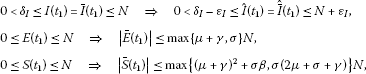(4.17)

where the coordinates change (3.3) and the fact that ${\beta }_{1}S\left({t}_{1}\right)\le \beta N$ has been used. By using continuity arguments of the functions $\overline{I}\left(t\right)$, $\stackrel{ˆ}{\overline{I}}\left(t\right)$, $\overline{E}\left(t\right)$ and $\overline{S}\left(t\right)$ $\mathrm{\forall }{t}_{1}\le t\le {t}_{f}$, there exists some definite-positive, monotone function ${T}_{I}={T}_{I}\left({\delta }_{I},{\epsilon }_{I}\right)$ increasing with ${\delta }_{I}$, decreasing with ${\epsilon }_{I}$ and satisfying ${lim}_{{\delta }_{I}\to \mathrm{\infty },{\epsilon }_{I}\to 0}\left\{{T}_{I}\left({\delta }_{I},{\epsilon }_{I}\right)\right\}=\mathrm{\infty }$, such that $0<{m}_{I}\le \overline{I}\left(t\right)\le {M}_{I}<\mathrm{\infty }$, $0<{m}_{I}^{\prime }\le \stackrel{ˆ}{\overline{I}}\left(t\right)\le {M}_{I}^{\prime }<\mathrm{\infty }$, $|\overline{E}\left(t\right)|\le {M}_{E}<\mathrm{\infty }$ and $|\overline{S}\left(t\right)|\le {M}_{S}<\mathrm{\infty }$ $\mathrm{\forall }t\in \left[{t}_{1},{t}_{1}+{T}_{I}\right)$ with ${m}_{I}^{\prime }<{\delta }_{I}^{\prime }$. Then one obtains that

$|\phi \left(\stackrel{ˆ}{\overline{x}}\left(t\right)\right)-\phi \left(\overline{x}\left(t\right)\right)|\le {K}_{I}|{\overline{e}}_{I}\left(t\right)|+{K}_{E}|{\overline{e}}_{E}\left(t\right)|+{K}_{S}|{\overline{e}}_{S}\left(t\right)|$
(4.18)

$\mathrm{\forall }t\in \left[{t}_{1},{t}_{1}+T\right)$, with $T\stackrel{\mathrm{\Delta }}{=}min\left\{{T}_{e},{T}_{I}\right\}$, by taking into account the definition of $\phi \left(\overline{x}\left(t\right)\right)$ in (3.5) and where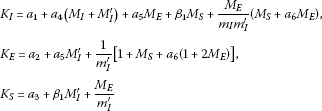(4.19)

with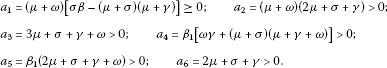(4.20)

Finally, the definition of ${t}_{1}$ also implies that ${u}_{l}\left({t}_{1}\right)={M}_{u}\ge {\epsilon }_{u}>0$. Then by using continuity arguments of the function ${u}_{l}\left(t\right)$ $\mathrm{\forall }t\in {\mathbb{R}}_{0+}$, or continuity arguments of $u\left(t\right)$ $\mathrm{\forall }{t}_{1}\le t\le {t}_{f}$, there exists some definite-positive, monotone function ${T}_{u}={T}_{u}\left({\epsilon }_{u}\right)$, increasing with ${\epsilon }_{u}$ and satisfying ${lim}_{{\epsilon }_{u}\to \mathrm{\infty }}\left\{{T}_{u}\left({\epsilon }_{u}\right)\right\}=\mathrm{\infty }$, such that $u\left(t\right)={u}_{l}\left(t\right)\ge 0$ $\mathrm{\forall }t\in \left[{t}_{1},{t}_{1}+{T}_{u}\right)$. Then from (4.14), (4.15) and (4.18)-(4.20), it follows that

$\begin{array}{rcl}\stackrel{˙}{W}\left(t\right)& \le & {\stackrel{˙}{W}}_{0}\left(t\right)+|{\stackrel{˙}{W}}_{\phi }\left(t\right)|+{\stackrel{˙}{W}}_{u}\left(t\right)\\ \le & {\stackrel{˙}{W}}_{0}\left(t\right)+{\theta }^{-3}|{\overline{e}}_{I}\left(t\right)-3{\theta }^{-1}{\overline{e}}_{E}\left(t\right)+6{\theta }^{-2}{\overline{e}}_{S}\left(t\right)|\left({K}_{I}|{\overline{e}}_{I}\left(t\right)|+{K}_{E}|{\overline{e}}_{E}\left(t\right)|+{K}_{S}|{\overline{e}}_{S}\left(t\right)|\right)\\ -\mu \sigma \beta {\theta }^{-3}\left[{\overline{e}}_{I}\left(t\right)-3{\theta }^{-1}{\overline{e}}_{E}\left(t\right)+6{\theta }^{-2}{\overline{e}}_{S}\left(t\right)\right]{\overline{e}}_{I}\left(t\right){u}_{l}\left(t\right)\le 0\phantom{\rule{1em}{0ex}}\mathrm{\forall }t\in \left[{t}_{1},{t}_{1}+{T}_{m}\right)\end{array}$
(4.21)

with ${T}_{m}\stackrel{\mathrm{\Delta }}{=}min\left\{T,{T}_{u}\right\}=min\left\{{T}_{e},{T}_{I},{T}_{u}\right\}$, if the observer gain θ is large enough for both ${\stackrel{˙}{W}}_{0}\left(t\right)\le 0$ and $\left[{\overline{e}}_{I}\left(t\right)-3{\theta }^{-1}{\overline{e}}_{E}\left(t\right)+6{\theta }^{-2}{\overline{e}}_{S}\left(t\right)\right]{\overline{e}}_{I}\left(t\right)\ge 0$ to be guaranteed $\mathrm{\forall }t\in \left[{t}_{1},{t}_{1}+{T}_{m}\right)$. In this context, on the one hand, note that all terms of ${\stackrel{˙}{W}}_{0}\left(t\right)$ in (4.15), except the one with $\frac{9}{4}{\theta }^{-2}{\overline{e}}_{E}^{2}$, are strictly negative whenever ${\overline{e}}_{I}\left(t\right)\ne 0$, ${\overline{e}}_{E}\left(t\right)\ne 0$ and ${\overline{e}}_{S}\left(t\right)\ne 0$ for any observer gain $\theta >0$. Such a term $\frac{9}{4}{\theta }^{-2}{\overline{e}}_{E}^{2}$ may be made small enough, in comparison with the absolute value of the contribution of the rest of the terms in ${\stackrel{˙}{W}}_{0}\left(t\right)$, by means of a large enough observer gain $\theta >0$ so that ${\stackrel{˙}{W}}_{0}\left(t\right)\le 0$ $\mathrm{\forall }t\in \left[{t}_{1},{t}_{1}+{T}_{m}\right)$. On the other hand, $\left[{\overline{e}}_{I}\left(t\right)-3{\theta }^{-1}{\overline{e}}_{E}\left(t\right)+6{\theta }^{-2}{\overline{e}}_{S}\left(t\right)\right]{\overline{e}}_{I}\left(t\right)\ge 0$ can be also ensured with a large enough observer gain $\theta >0$. In this case, the term depending on ${u}_{l}\left(t\right)$ in (4.21) is strictly negative $\mathrm{\forall }t\in \left[{t}_{1},{t}_{1}+{T}_{m}\right)$ since ${u}_{l}\left(t\right)\ge 0$ in such a time interval. Note also that ${\theta }^{-3}|{\overline{e}}_{I}\left(t\right)-3{\theta }^{-1}{\overline{e}}_{E}\left(t\right)+6{\theta }^{-2}{\overline{e}}_{S}\left(t\right)|\left({K}_{I}|{\overline{e}}_{I}\left(t\right)|+{K}_{E}|{\overline{e}}_{E}\left(t\right)|+{K}_{S}|{\overline{e}}_{S}\left(t\right)|\right)$, which is non-negative $\mathrm{\forall }t\in \left[{t}_{1},{t}_{1}+{T}_{m}\right)$, may be made small in comparison with the absolute value of the rest of the terms in (4.21) by using a sufficiently large $\theta >0$ so that $\stackrel{˙}{W}\left(t\right)\le 0$ $\mathrm{\forall }t\in \left[{t}_{1},{t}_{1}+{T}_{m}\right)$.

From (4.21) and the definition of the Lyapunov function candidate $W\left(t\right)$, if follows that $\parallel \overline{e}\left(t\right)\parallel$ is monotone decreasing $\mathrm{\forall }t\in \left[{t}_{1},{t}_{1}+{T}_{m}\right)$ and that $|{\overline{e}}_{I}\left(t\right)|$ is also monotone decreasing $\mathrm{\forall }t\in \left[{t}_{1},{t}_{1}+{T}_{m}\right)$ with $|{\overline{e}}_{I}\left({t}_{1}\right)|={\epsilon }_{I}$ from the definition of the time instant ${t}_{1}$. Then from (4.16), it follows that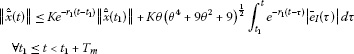(4.22)

since ${r}_{1}>0$ is the absolute value of the dominant eigenvalue of the state matrix A in (4.16), according to condition (vi) of the theorem, and where $K\ge 1$ is some upper-bound of the transition matrix associated to the system (4.16). Moreover, $|{\overline{e}}_{I}\left(t\right)|\le {\overline{\epsilon }}_{I}{e}^{-\rho \left(t-{t}_{1}\right)}$ $\mathrm{\forall }t\in \left[{t}_{1},{t}_{1}+{T}_{m}\right)$ for some real constants ${\overline{\epsilon }}_{I}>{\epsilon }_{I}>0$ and $\rho >0$ from the fact that $|{\overline{e}}_{I}\left(t\right)|$ is monotone decreasing $\mathrm{\forall }t\in \left[{t}_{1},{t}_{1}+{T}_{m}\right)$. The constant $\rho >0$ is related to the observer gain $\theta >0$ in the sense that ρ can be as large as θ. Then from (4.22), one obtains that

$\begin{array}{rcl}\parallel \stackrel{ˆ}{\overline{x}}\left(t\right)\parallel & \le & K{e}^{-{r}_{1}\left(t-{t}_{1}\right)}\parallel \stackrel{ˆ}{\overline{x}}\left({t}_{1}\right)\parallel +{K}^{\prime }{\overline{\epsilon }}_{I}{e}^{-{r}_{1}t}{e}^{-\rho {t}_{1}}{\int }_{{t}_{1}}^{t}{e}^{\left({r}_{1}-\rho \right)\tau }\phantom{\rule{0.2em}{0ex}}d\tau \\ =& K{e}^{-{r}_{1}\left(t-{t}_{1}\right)}\parallel \stackrel{ˆ}{\overline{x}}\left({t}_{1}\right)\parallel +\frac{{K}^{\prime }{\overline{\epsilon }}_{I}}{{r}_{1}-\rho }\left[{e}^{-\rho \left(t-{t}_{1}\right)}-{e}^{-{r}_{1}\left(t-{t}_{1}\right)}\right]\phantom{\rule{1em}{0ex}}\mathrm{\forall }{t}_{1}\le t<{t}_{1}+{T}_{m}\end{array}$
(4.23)

provided that $\rho \ne {r}_{1}$. Note that if $\rho ={r}_{1}$ satisfies $|{\overline{e}}_{I}\left(t\right)|\le {\overline{\epsilon }}_{I}{e}^{-\rho \left(t-{t}_{1}\right)}$ $\mathrm{\forall }t\in \left[{t}_{1},{t}_{1}+{T}_{m}\right)$, then any $0<\rho <{r}_{1}$ also satisfies it. From (4.23), the population estimates vector $\stackrel{ˆ}{\overline{x}}\left(t\right)$ would converge asymptotically to zero as $t\to \mathrm{\infty }$. As a consequence, if the time interval ${T}_{m}$ is sufficiently large for the conditions (4.17), (4.18) and ${u}_{l}\left(t\right)\ge 0$ $\mathrm{\forall }t\in \left[{t}_{1},{t}_{1}+{T}_{m}\right)$, (4.21) be satisfied until reaching a time instant ${t}_{f}$ at which $\stackrel{ˆ}{\overline{I}}\left({t}_{f}\right)=\stackrel{ˆ}{I}\left({t}_{f}\right)\le {\delta }_{I}^{\prime }$, then the propagation of the infection will be eradicated from the population by taking into account that ${\overline{e}}_{I}\left({t}_{f}\right)=\stackrel{ˆ}{\overline{I}}\left({t}_{f}\right)-\overline{I}\left({t}_{f}\right)$ is very small, while maintaining the positivity of the controlled SEIR model. □

Remarks 4.1 (i) The observer dynamics consists of a copy of that of the system model in the transformed state representation $\overline{x}\left(t\right)={\left[\overline{I}\left(t\right)\phantom{\rule{0.25em}{0ex}}\overline{E}\left(t\right)\phantom{\rule{0.25em}{0ex}}\overline{S}\left(t\right)\right]}^{T}$ with an additional term proportional to the observation error $\stackrel{ˆ}{\overline{y}}\left(t\right)-y\left(t\right)=C\stackrel{ˆ}{\overline{x}}\left(t\right)-y\left(t\right)$. Moreover, such a corrective term does not depend on the system realization, but only on the dimension of the state space since $C\stackrel{ˆ}{\overline{x}}\left(t\right)=\stackrel{ˆ}{\overline{I}}\left(t\right)=\stackrel{ˆ}{I}\left(t\right)=C\stackrel{ˆ}{x}\left(t\right)$ according to the coordinate transformation (3.3).

1. (ii)

The control strategy described by (4.1)-(4.3) is composed of two consecutive stages. The first one is an observation stage at which no control action is applied to the SEIR model and the main objective is that the observer variables go converging to the true partial populations. Once the estimation error is sufficiently small, such that the observer variables track the true population with a suitable precision, this observation stage ends. Then the second stage at which a control action is applied begins. Such a control action linearizes the closed-loop observer so that the estimated variables converge asymptotically to zero as time grows while also guaranteeing the convergence to zero of the estimation error. This implies that the true infectious and infected populations decrease toward zero as time grows until reaching a finite time instant ${t}_{f}$ when the epidemic disease is considered eradicated from the host population. After such an instant, the control action is removed from the system.

2. (iii)

On the one hand, the length of the observation stage may be short enough with a suitable choice of the observer gain. In this sense, such a length decreases as the value of the observer gain θ increases. Then a large value for θ seems to be appropriate. On the other hand, a large value of θ makes the contribution of the perturbation term ${\left[3\theta \phantom{\rule{0.25em}{0ex}}3{\theta }^{2}\phantom{\rule{0.25em}{0ex}}{\theta }^{3}\right]}^{T}{\overline{e}}_{I}\left(t\right)$ in (4.16) considerable. As a consequence, a tradeoff value for θ has to be searched. In this context, in the limiting case that θ was a very small positive real number (i.e. $\theta \to {0}^{+}$) the closed-loop dynamics of the observer system would be $\stackrel{˙}{\stackrel{ˆ}{\overline{x}}}=A\stackrel{ˆ}{\overline{x}}$ with A defined in (3.11), i.e. such a dynamics would be identical to that of the closed loop SEIR model without the observer. As a consequence, if the controller parameters ${\lambda }_{i}$ for $i\in \left\{0,1,2\right\}$ were chosen according to the conditions of Theorem 3.3, the estimates in $\stackrel{ˆ}{x}\left(t\right)$ would be non-negative $\mathrm{\forall }t\in {\mathbb{R}}_{0+}$ and